Abstract

This is the companion vignette to the ‘Using R and Bioconductor for proteomics data analysis’ manuscript that presents an overview of R and Bioconductor software for mass spectrometry and proteomics data. It provides the code to reproduce the figures in the paper.

## Introduction

This vignette illustrates existing and Bioconductor infrastructure for the visualisation of mass spectrometry and proteomics data. The code details the visualisations presented in

Gatto L, Breckels LM, Naake T, Gibb S. Visualisation of proteomics data using R and Bioconductor. Proteomics. 2015 Feb 18. doi: 10.1002/pmic.201400392. PubMed PMID: 25690415.

NB: I you are interested in R packages for mass spectrometry-based proteomics and metabolomics, see also the R for Mass Spectrometry initiative packages and the tutorial book

## Ascombe’s quartet

x1 x2 x3 x4 y1 y2 y3 y4
10 10 10 8 8.04 9.14 7.46 6.58
8 8 8 8 6.95 8.14 6.77 5.76
13 13 13 8 7.58 8.74 12.74 7.71
9 9 9 8 8.81 8.77 7.11 8.84
11 11 11 8 8.33 9.26 7.81 8.47
14 14 14 8 9.96 8.10 8.84 7.04
6 6 6 8 7.24 6.13 6.08 5.25
4 4 4 19 4.26 3.10 5.39 12.50
12 12 12 8 10.84 9.13 8.15 5.56
7 7 7 8 4.82 7.26 6.42 7.91
5 5 5 8 5.68 4.74 5.73 6.89
tab <- matrix(NA, 5, 4)
colnames(tab) <- 1:4
rownames(tab) <- c("var(x)", "mean(x)",
"var(y)", "mean(y)",
"cor(x,y)")

for (i in 1:4)
tab[, i] <- c(var(anscombe[, i]),
mean(anscombe[, i]),
var(anscombe[, i+4]),
mean(anscombe[, i+4]),
cor(anscombe[, i], anscombe[, i+4]))
1 2 3 4
var(x) 11.0000000 11.0000000 11.0000000 11.0000000
mean(x) 9.0000000 9.0000000 9.0000000 9.0000000
var(y) 4.1272691 4.1276291 4.1226200 4.1232491
mean(y) 7.5009091 7.5009091 7.5000000 7.5009091
cor(x,y) 0.8164205 0.8162365 0.8162867 0.8165214

While the residuals of the linear regression clearly indicate fundamental differences in these data, the most simple and straightforward approach is visualisation to highlight the fundamental differences in the datasets.

ff <- y ~ x

mods <- setNames(as.list(1:4), paste0("lm", 1:4))

par(mfrow = c(2, 2), mar = c(4, 4, 1, 1))
for (i in 1:4) {
ff[2:3] <- lapply(paste0(c("y","x"), i), as.name)
plot(ff, data = anscombe, pch = 19, xlim = c(3, 19), ylim = c(3, 13))
mods[[i]] <- lm(ff, data = anscombe)
abline(mods[[i]])
}lm1 lm2 lm3 lm4
0.0390000 1.1390909 -0.5397273 -0.421
-0.0508182 1.1390909 -0.2302727 -1.241
-1.9212727 -0.7609091 3.2410909 0.709
1.3090909 1.2690909 -0.3900000 1.839
-0.1710909 0.7590909 -0.6894545 1.469
-0.0413636 -1.9009091 -1.1586364 0.039
1.2393636 0.1290909 0.0791818 -1.751
-0.7404545 -1.9009091 0.3886364 0.000
1.8388182 0.1290909 -0.8491818 -1.441
-1.6807273 0.7590909 -0.0805455 0.909
0.1794545 -0.7609091 0.2289091 -0.111

## The MA plot example

The following code chunk connects to the PXD000001 data set on the ProteomeXchange repository and fetches the mzTab file. After missing values filtering, we extract relevant data (log2 fold-changes and log10 mean expression intensities) into data.frames.

library("rpx")
px1 <- PXDataset("PXD000001")
## Loading PXD000001 from cache.
mztab <- pxget(px1, "F063721.dat-mztab.txt")
## Loading F063721.dat-mztab.txt from cache.
library("MSnbase")
## here, we need to specify the (old) mzTab version 0.9
qnt <- readMzTabData(mztab, what = "PEP", version = "0.9")
sampleNames(qnt) <- reporterNames(TMT6)
qnt <- filterNA(qnt)
## may be combineFeatuers

spikes <- c("P02769", "P00924", "P62894", "P00489")
protclasses <- as.character(fData(qnt)$accession) protclasses[!protclasses %in% spikes] <- "Background" madata42 <- data.frame(A = rowMeans(log(exprs(qnt[, c(4, 2)]), 10)), M = log(exprs(qnt)[, 4], 2) - log(exprs(qnt)[, 2], 2), data = rep("4vs2", nrow(qnt)), protein = fData(qnt)$accession,
class = factor(protclasses))

madata62 <- data.frame(A = rowMeans(log(exprs(qnt[, c(6, 2)]), 10)),
M = log(exprs(qnt)[, 6], 2) - log(exprs(qnt)[, 2], 2),
data = rep("6vs2", nrow(qnt)),
protein = fData(qnt)$accession, class = factor(protclasses)) madata <- rbind(madata42, madata62) ### The traditional plotting system par(mfrow = c(1, 2)) plot(M ~ A, data = madata42, main = "4vs2", xlab = "A", ylab = "M", col = madata62$class)
plot(M ~ A, data = madata62, main = "6vs2",
xlab = "A", ylab = "M", col = madata62$class)### lattice library("lattice") latma <- xyplot(M ~ A | data, data = madata, groups = madata$class,
auto.key = TRUE)
print(latma)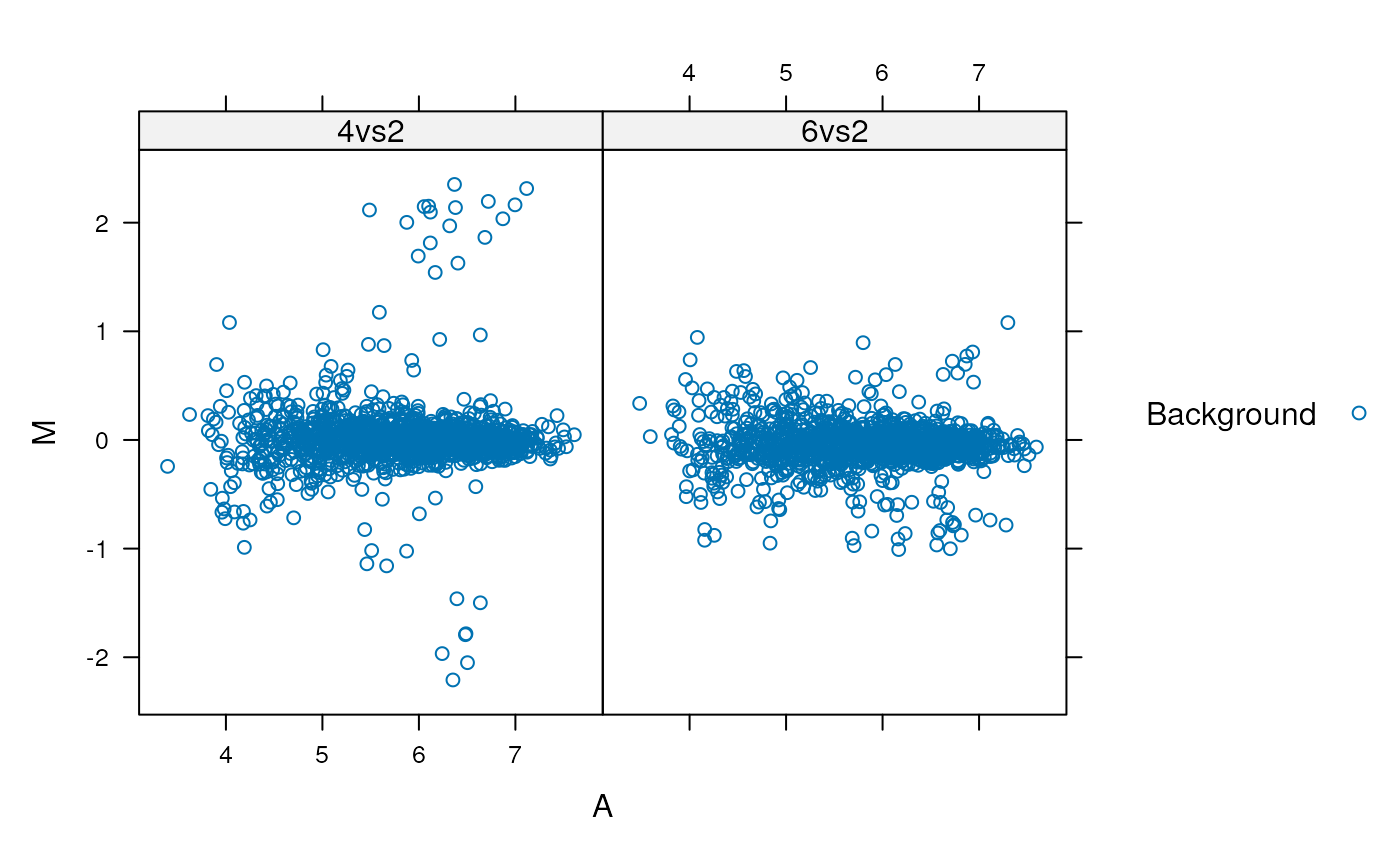### ggplot2

library("ggplot2")
ggma <- ggplot(aes(x = A, y = M, colour = class), data = madata,
colour = class) +
geom_point() +
facet_grid(. ~ data)
print(ggma)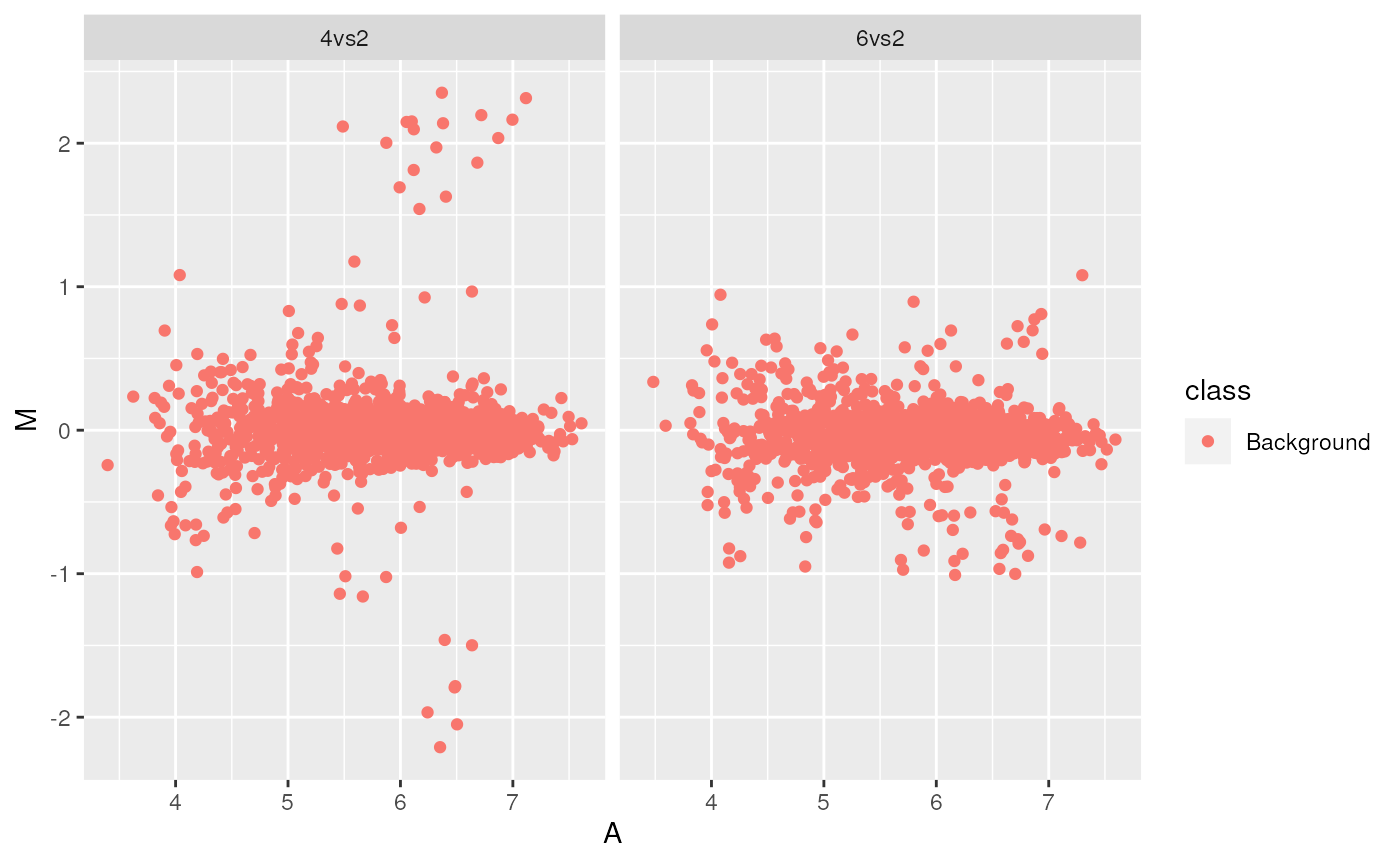### Customization

library("RColorBrewer")
bcols <- brewer.pal(4, "Set1")
cls <- c("Background" = "#12121230",
"P02769" = bcols,
"P00924" = bcols,
"P62894" = bcols,
"P00489" = bcols)
ggma2 <- ggplot(aes(x = A, y = M, colour = class),
data = madata) + geom_point(shape = 19) +
facet_grid(. ~ data) + scale_colour_manual(values = cls) +
guides(colour = guide_legend(override.aes = list(alpha = 1)))
print(ggma2)### The MAplot method for MSnSet instances

MAplot(qnt, cex = .8)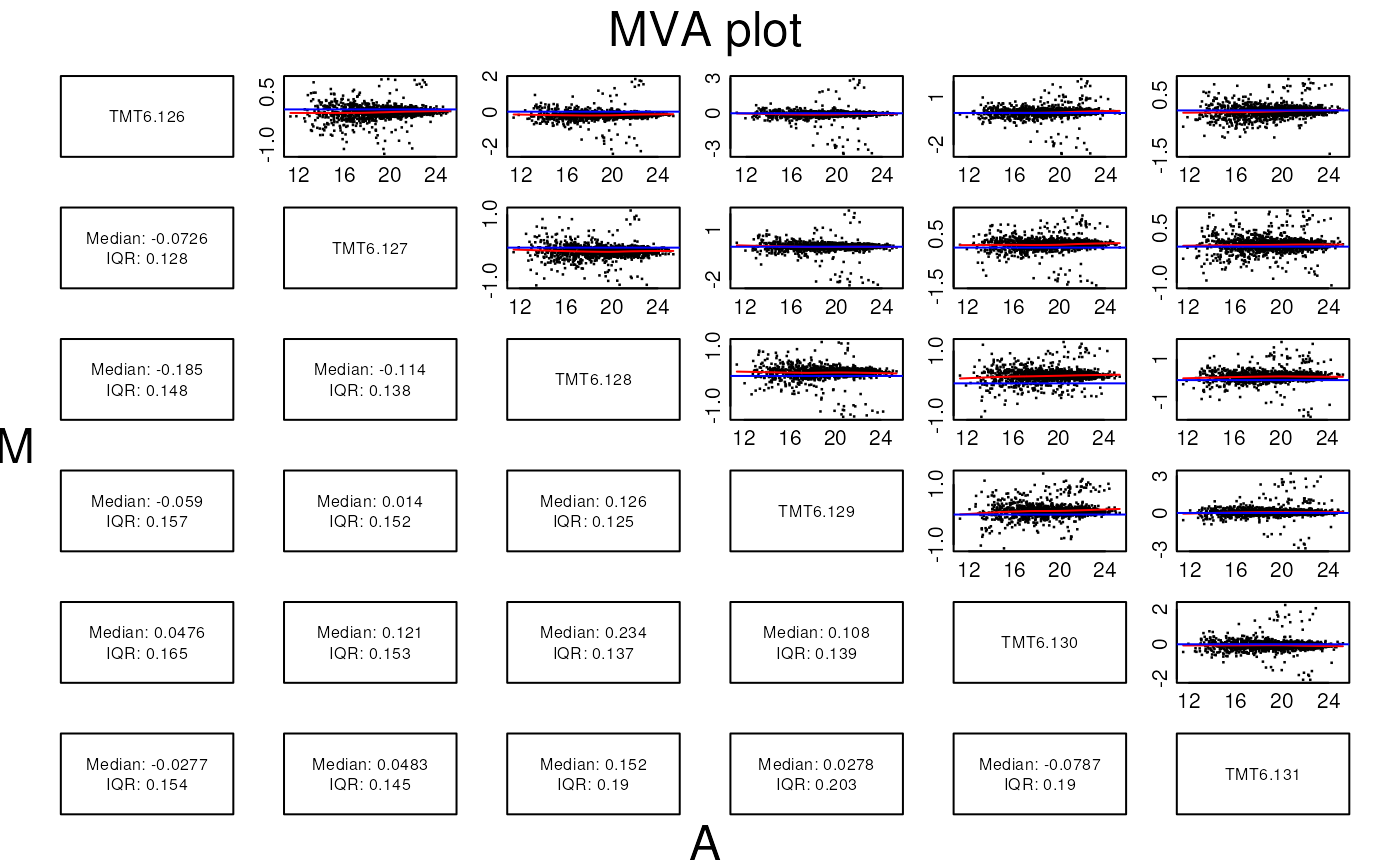### An interactive shiny app for MA plots

This (now outdated and deprecated) app is based on Mike Love’s shinyMA application, adapted for a proteomics data. A screen shot is displayed below.

See the excellent shiny web page for tutorials and the Mastering Shiny book for details on shiny.

### Volcano plots

Below, using the msmsTest package, we load a example MSnSet data with spectral couting data (from the r Biocpkg("msmsEDA") package) and run a statistical test to obtain (adjusted) p-values and fold-changes.

library("msmsEDA")
library("msmsTests")
data(msms.dataset)
## Pre-process expression matrix
e <- pp.msms.data(msms.dataset)
## Models and normalizing condition
null.f <- "y~batch"
alt.f <- "y~treat+batch"
div <- apply(exprs(e), 2, sum)
## Test
res <- msms.glm.qlll(e, alt.f, null.f, div = div)
lst <- test.results(res, e, pData(e)$treat, "U600", "U200 ", div, alpha = 0.05, minSpC = 2, minLFC = log2(1.8), method = "BH") Here, we produce the volcano plot by hand, with the plot function. In the second plot, we limit the x axis limits and add grid lines. plot(lst$tres$LogFC, -log10(lst$tres$p.value))plot(lst$tres$LogFC, -log10(lst$tres$p.value), xlim = c(-3, 3)) grid()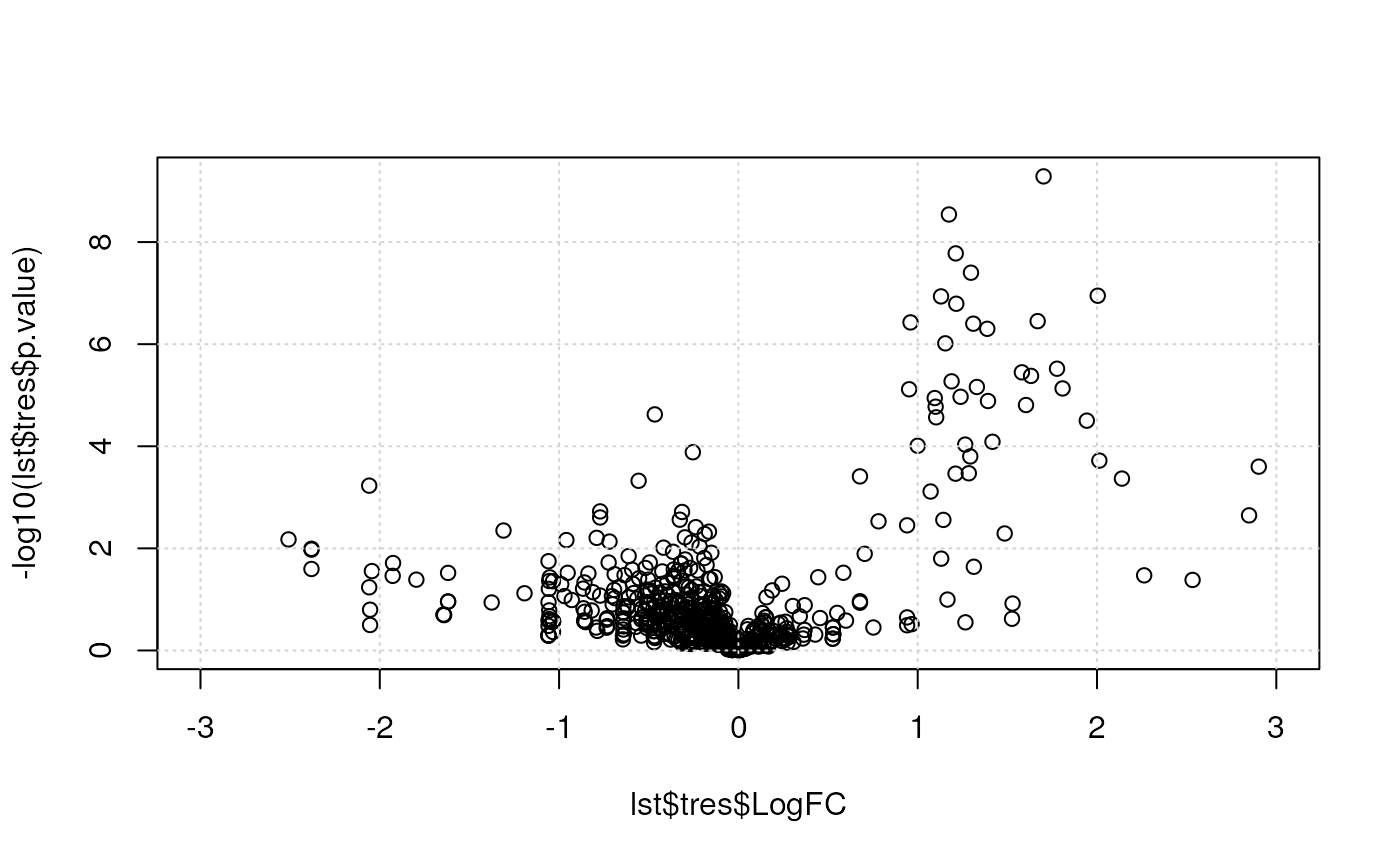Below, we use the res.volcanoplot function from the r Biocpkg("msmsTests") package. This functions uses the sample annotation stored with the quantitative data in the MSnSet object to colour the samples according to their phenotypes. ## Plot res.volcanoplot(lst$tres,
max.pval = 0.05,
min.LFC = 1,
maxx = 3,
maxy = NULL,
ylbls = 4)### A PCA plot

Using the counts.pca function from the msmsEDA package:

library("msmsEDA")
data(msms.dataset)
msnset <- pp.msms.data(msms.dataset)
lst <- counts.pca(msnset, wait = FALSE)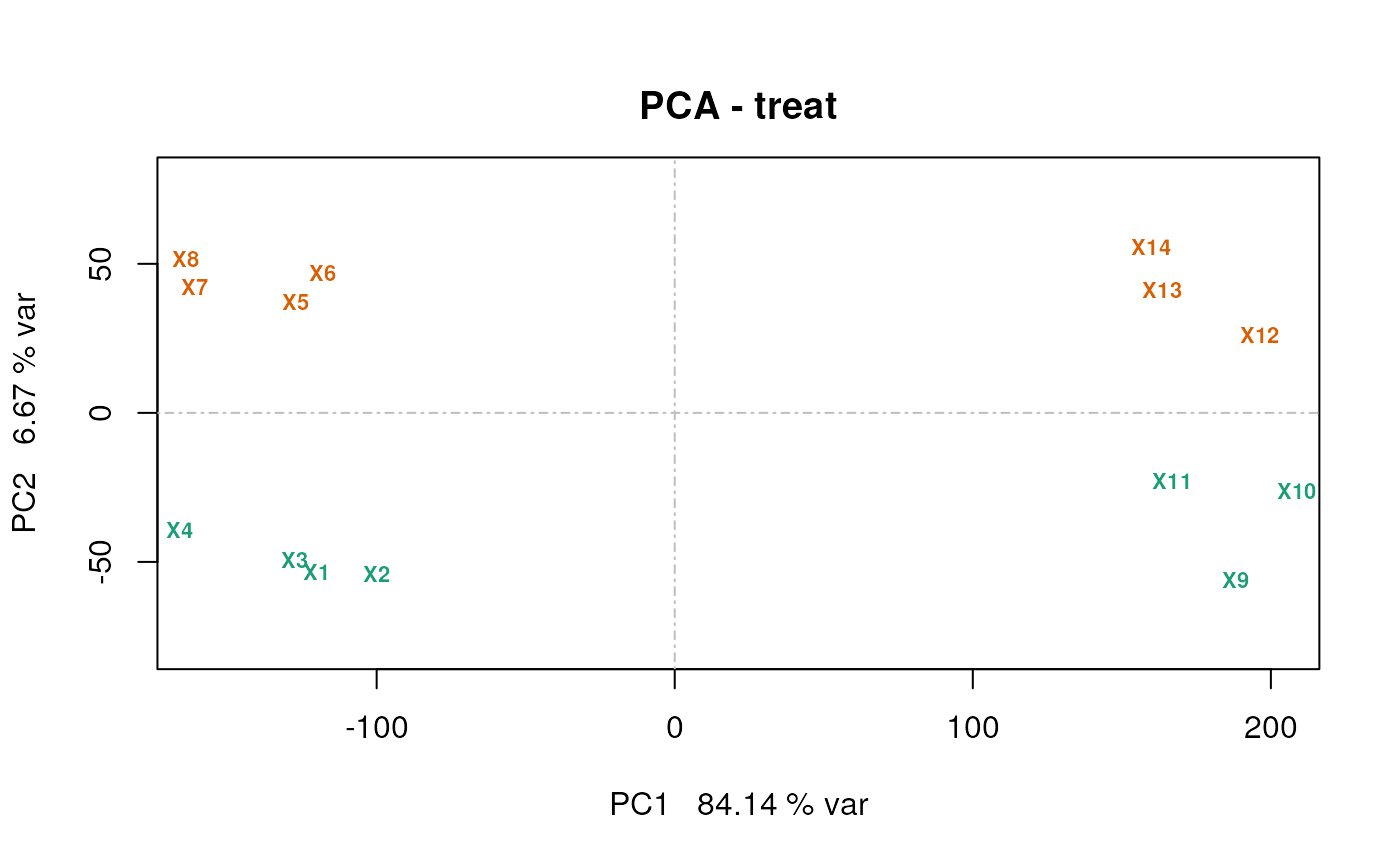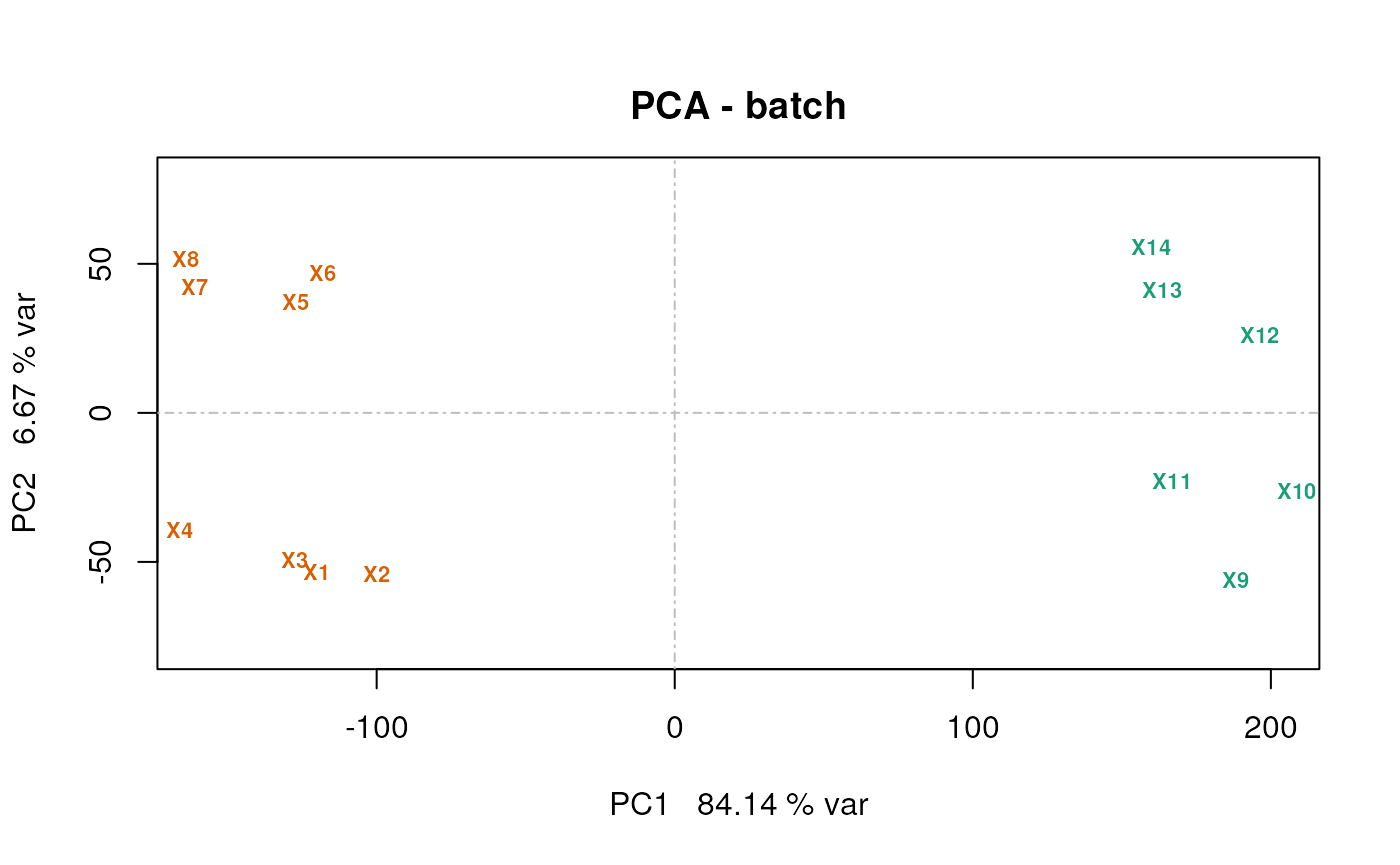It is also possible to generate the PCA data using the prcomp. Below, we extract the coordinates of PC1 and PC2 from the counts.pca result and plot them using the plot function.

pcadata <- lst$pca$x[, 1:2]
head(pcadata)
##                  PC1       PC2
## U2.2502.1 -120.26080 -53.55270
## U2.2502.2  -99.90618 -53.89979
## U2.2502.3 -127.35928 -49.29906
## U2.2502.4 -166.04611 -39.27557
## U6.2502.1 -127.18423  37.11614
## U6.2502.2 -117.97016  47.03702
plot(pcadata[, 1], pcadata[, 2],
xlab = "PCA1", ylab = "PCA2")
grid()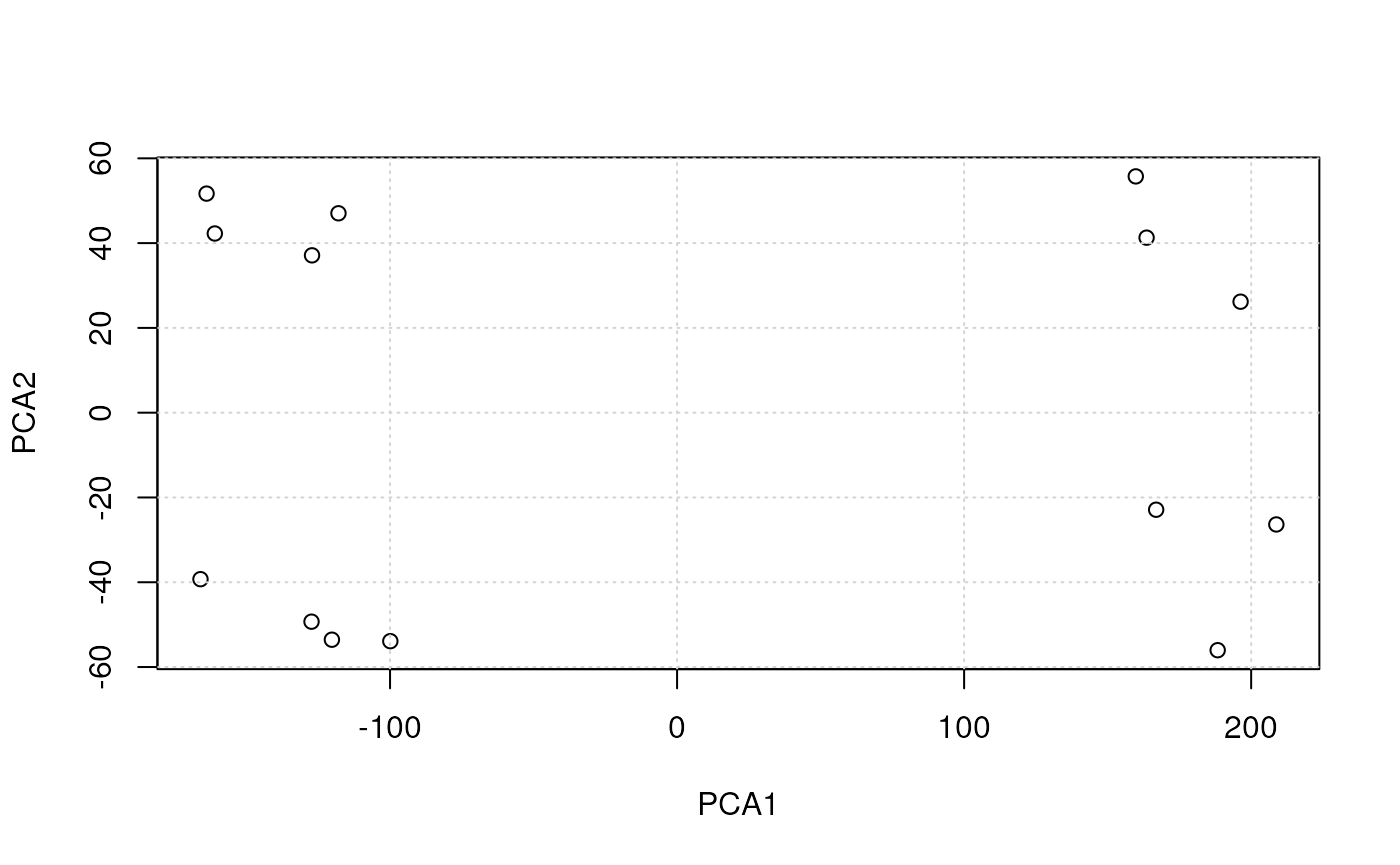## Plotting with R

kable(plotfuns)
scatterplots plot xyplot geom_point
histograms hist histgram geom_histogram
density plots plot(density()) densityplot geom_density
boxplots boxplot bwplot geom_boxplot
violin plots vioplot::vioplot bwplot(…, panel = panel.violin) geom_violin
line plots plot, matplot xyploy, parallelplot geom_line
bar plots barplot barchart geom_bar
pie charts pie geom_bar with polar coordinates
dot plots dotchart dotplot geom_point
stip plots stripchart stripplot goem_point
dendrogramms plot(hclust()) latticeExtra package ggdendro package
heatmaps image, heatmap levelplot geom_tile

Below, we are going to use a data from the pRolocdata to illustrate the plotting functions.

library("pRolocdata")
data(tan2009r1)

### Scatter plots

See the MA and volcano plot examples.

The default plot type is p, for points. Other important types are l for lines and h for histogram (see below).

### Historams and density plots

We extract the (normalised) intensities of the first sample

x <- exprs(tan2009r1)[, 1]

and plot the distribution with a histogram and a density plot next to each other on the same figure (using the mfrow par plotting paramter)

par(mfrow = c(1, 2))
hist(x)
plot(density(x))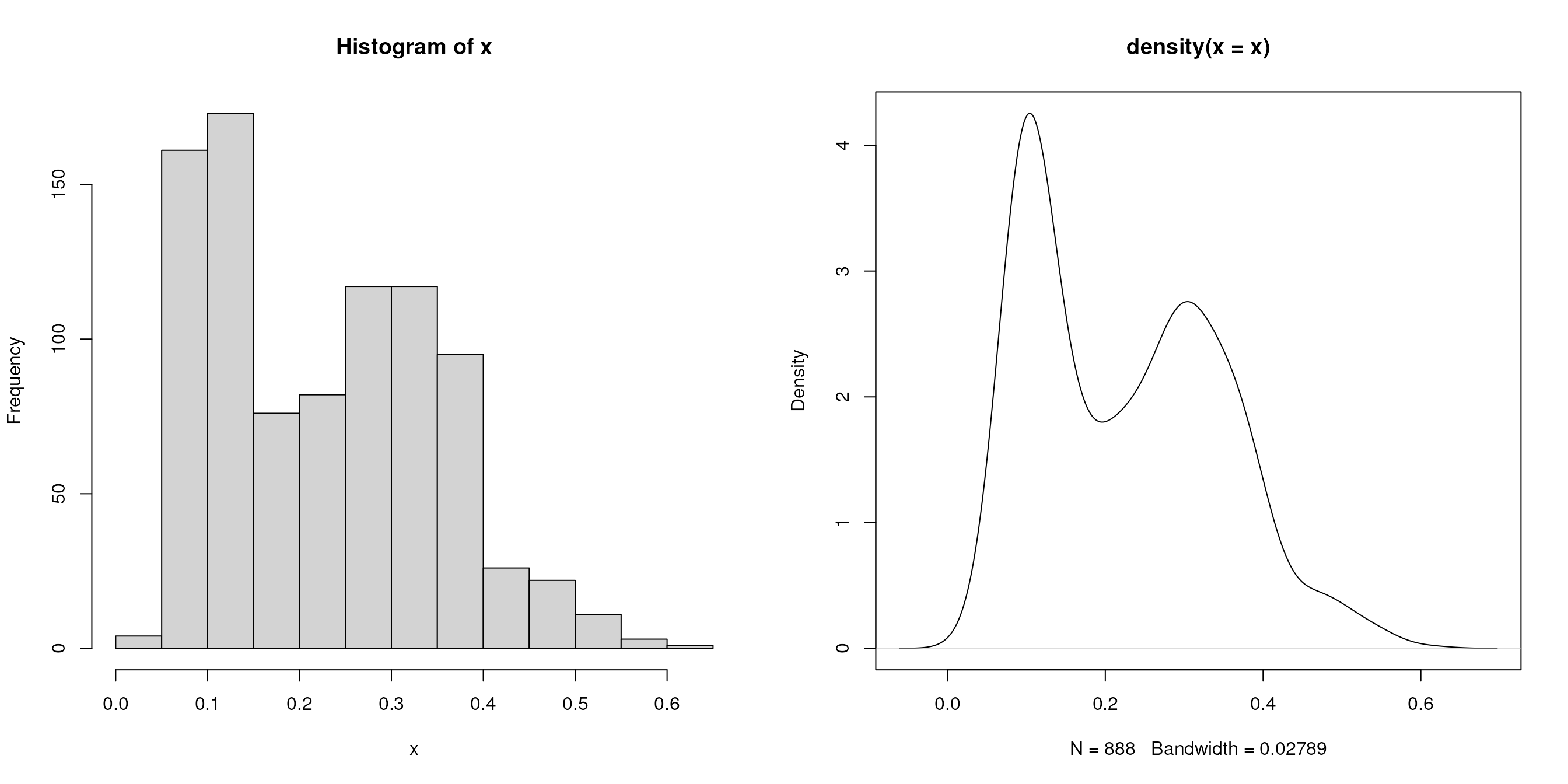### Box plots and violin plots

we first extract the 888 proteins by r ncol(tan2009r1) samples data matrix and plot the sample distributions next to each other using boxplot and beanplot (from the beanplot package).

library("beanplot")
x <- exprs(tan2009r1)
par(mfrow = c(2, 1))
boxplot(x)
beanplot(x[, 1], x[, 2], x[, 3], x[, 4], log = "")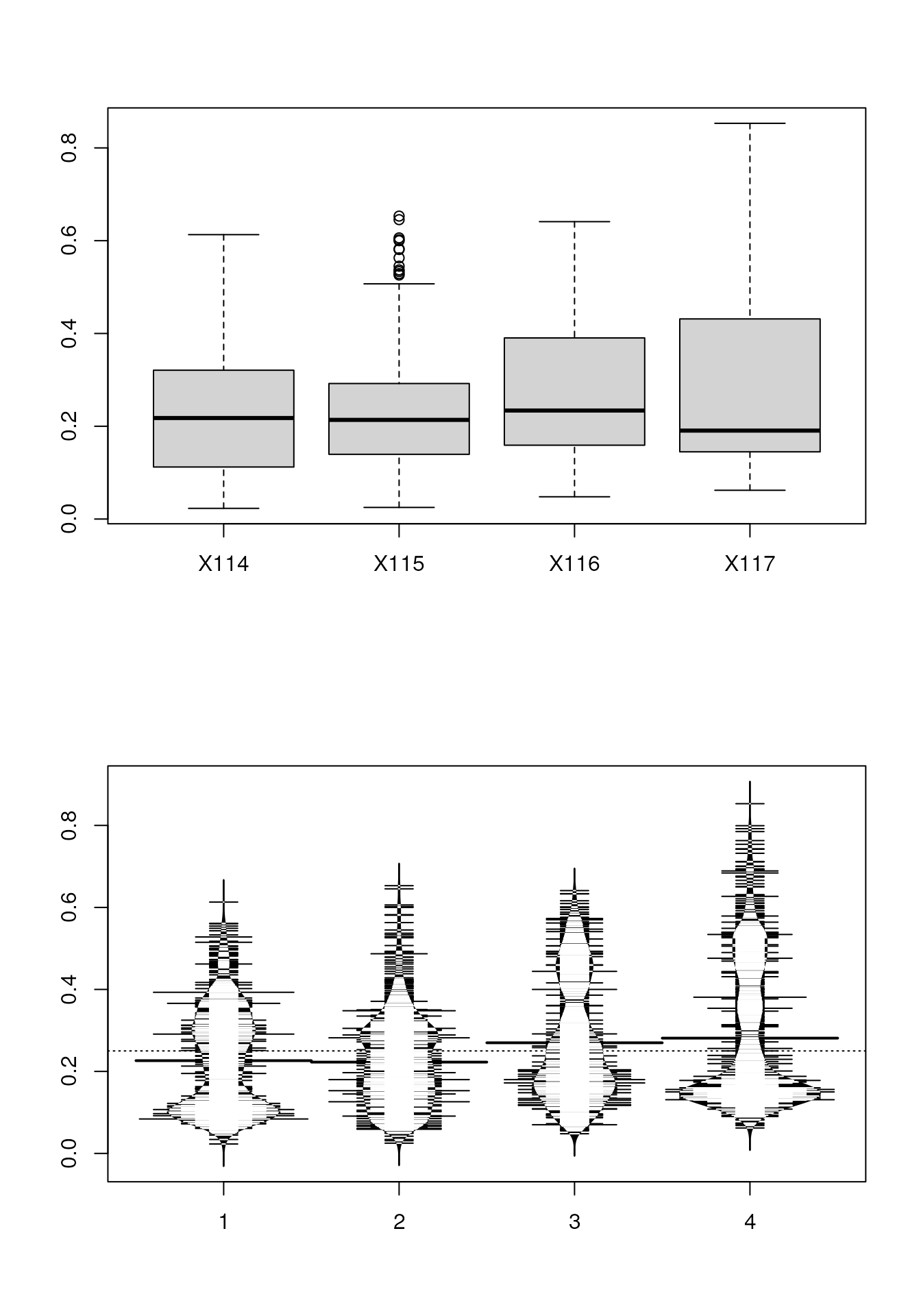### Line plots

below, we produce line plots that describe the protein quantitative profiles for two sets of proteins, namely er and mitochondrial proteins using matplot.

we need to transpose the matrix (with t) and set the type to both (b), to display points and lines, the colours to red and steel blue, the point characters to 1 (an empty point) and the line type to 1 (a solid line).

er <- fData(tan2009r1)$markers == "ER" mt <- fData(tan2009r1)$markers == "mitochondrion"

par(mfrow = c(2, 1))
matplot(t(x[er, ]), type = "b", col = "red", pch = 1, lty = 1)
matplot(t(x[mt, ]), type = "b", col = "steelblue", pch = 1, lty = 1)In the last section, about spatial proteomics, we use the specialised plotDist function from the pRoloc package to generate such figures.

### Bar and dot charts

To illustrate bar and dot charts, we cound the number of proteins in the respective class using table.

x <- table(fData(tan2009r1)$markers) x ## ## Cytoskeleton ER Golgi Lysosome mitochondrion ## 7 28 13 8 29 ## Nucleus Peroxisome PM Proteasome Ribosome 40S ## 21 4 34 15 20 ## Ribosome 60S unknown ## 32 677 par(mfrow = c(1, 2)) barplot(x) dotchart(as.numeric(x))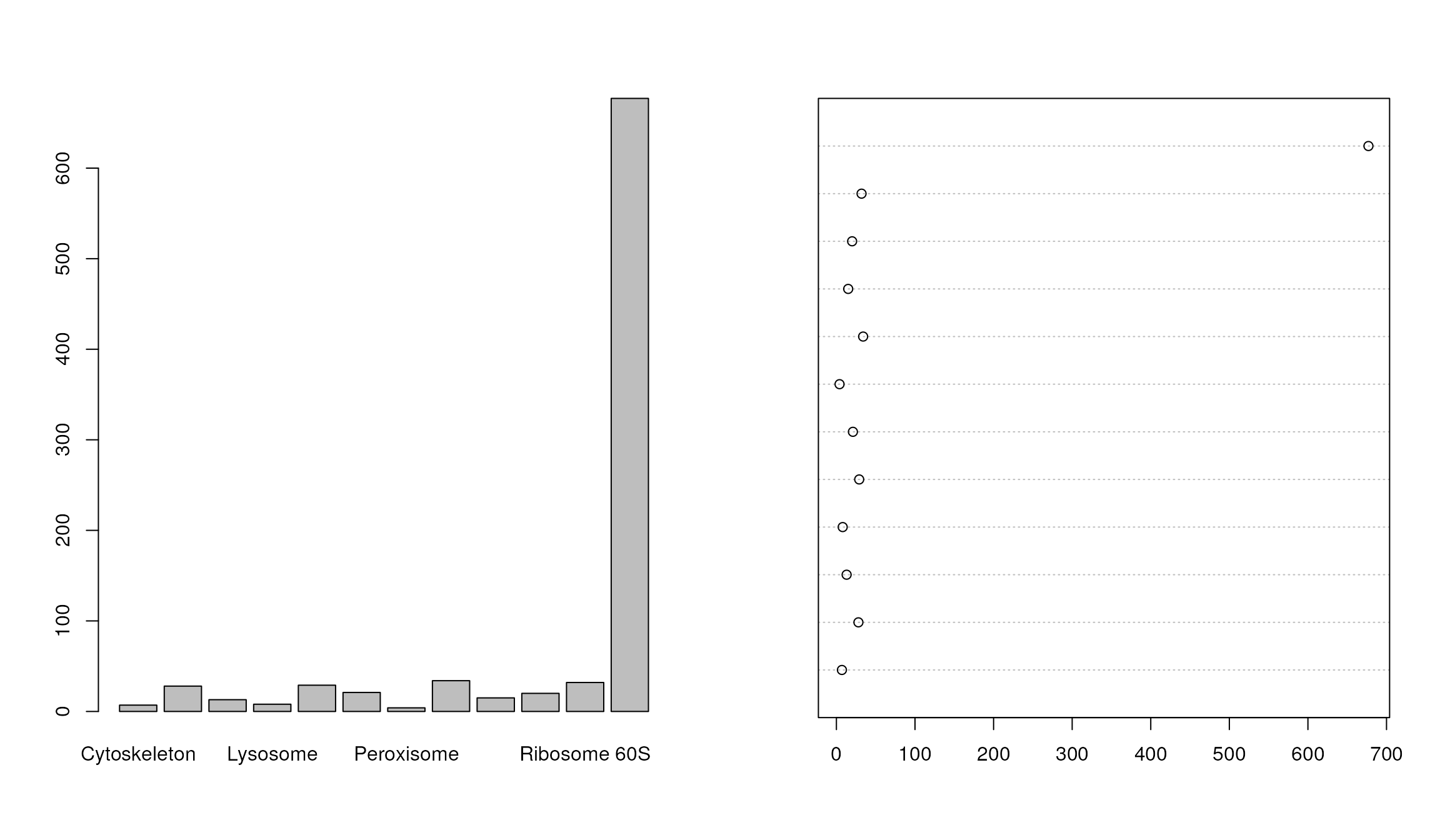### Heatmaps The easiest to produce a complete heatmap is with the heatmap function: heatmap(exprs(tan2009r1))To produce the a heatmap without the dendrograms, one can use the image function on a matrix or the specialised version for MSnSet objects from the MSnbase package. par(mfrow = c(1, 2)) x <- matrix(1:9, ncol = 3) image(x) image(tan2009r1)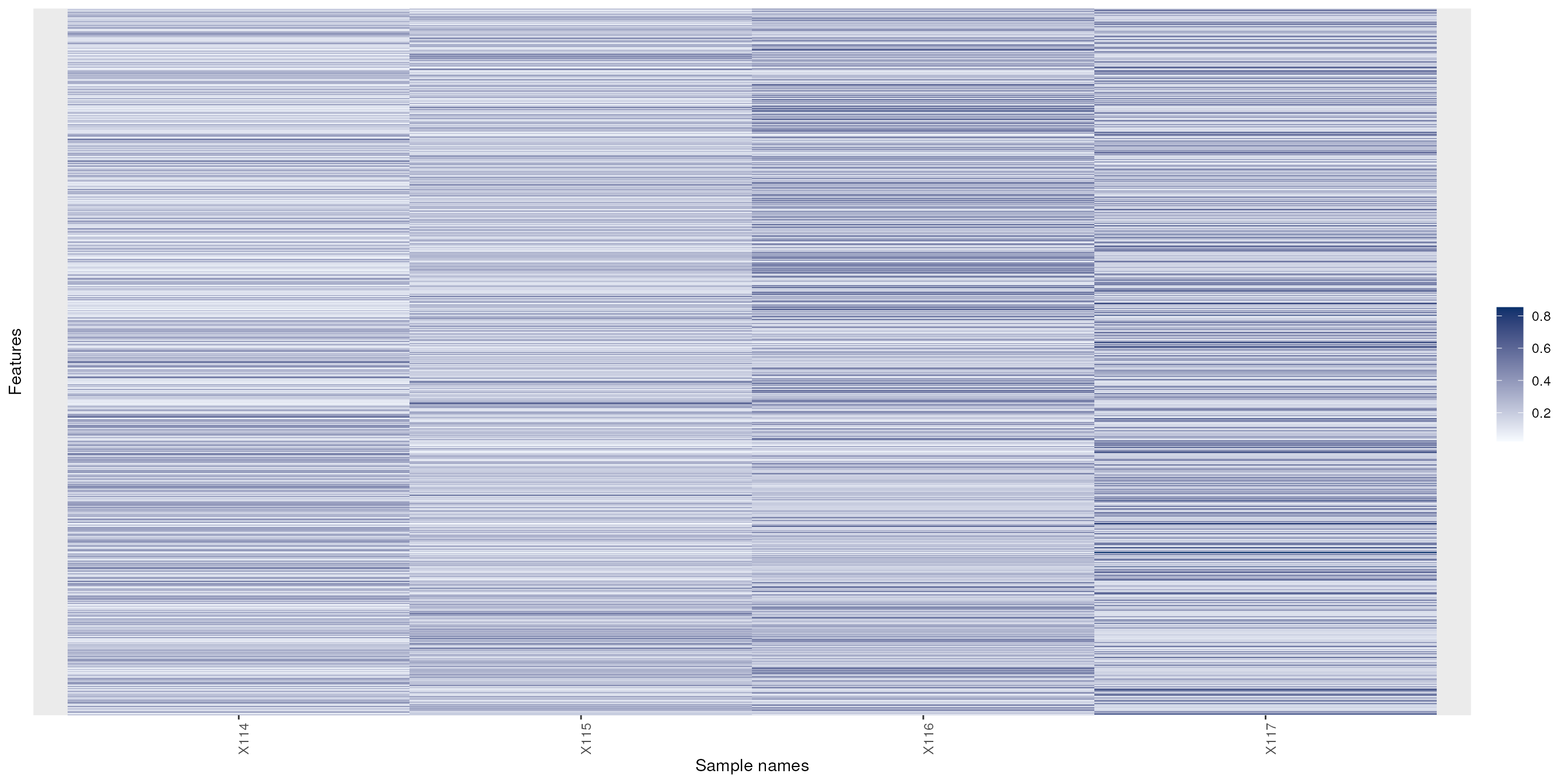See also gplots’s heatmap.2 function and the Heatplus Bioconductor package for more advanced heatmaps and the corrplot package for correlation matrices. ### Dendrograms The easiest way to produce and plot a dendrogram is: d <- dist(t(exprs(tan2009r1))) ## distance between samples hc <- hclust(d) ## hierarchical clustering plot(hc) ## visualisationSee also dendextend and this post to illustrate latticeExtra and ggdendro. ### Venn diagrams ## Visualising mass spectrometry data ### Direct access to the raw data library("mzR") mzf <- pxget(px1, "TMT_Erwinia_1uLSike_Top10HCD_isol2_45stepped_60min_01-20141210.mzML") ## Loading TMT_Erwinia_1uLSike_Top10HCD_isol2_45stepped_60min_01-20141210.mzML from cache. ms <- openMSfile(mzf) hd <- header(ms) ms1 <- which(hd$msLevel == 1)

rtsel <- hd$retentionTime[ms1] / 60 > 30 & hd$retentionTime[ms1] / 60 < 35
lout <- matrix(NA, ncol = 10, nrow = 8)
lout[1:2, ] <- 1
for (ii in 3:4)
lout[ii, ] <- c(2, 2, 2, 2, 2, 2, 3, 3, 3, 3)
lout[5, ] <- rep(4:8, each = 2)
lout[6, ] <- rep(4:8, each = 2)
lout[7, ] <- rep(9:13, each = 2)
lout[8, ] <- rep(9:13, each = 2)
i <- ms1[which(rtsel)]
j <- ms1[which(rtsel)]
ms2 <- (i+1):(j-1)
layout(lout)
par(mar=c(4,2,1,1))
plot(chromatogram(ms)[], type = "l")
abline(v = hd[i, "retentionTime"], col = "red")
par(mar = c(3, 2, 1, 0))
plot(peaks(ms, i), type = "l", xlim = c(400, 1000))
legend("topright", bty = "n",
legend = paste0(
"Acquisition ", hd[i, "acquisitionNum"],  "\n",
"Retention time ", formatRt(hd[i, "retentionTime"])))
abline(h = 0)
abline(v = hd[ms2, "precursorMZ"],
col = c("#FF000080",
rep("#12121280", 9)))
par(mar = c(3, 0.5, 1, 1))
plot(peaks(ms, i), type = "l", xlim = c(521, 522.5), yaxt = "n")
abline(h = 0)
abline(v = hd[ms2, "precursorMZ"], col = "#FF000080")
par(mar = c(2, 2, 0, 1))
for (ii in ms2) {
p <- peaks(ms, ii)
plot(p, xlab = "", ylab = "", type = "h", cex.axis = .6)
legend("topright",
legend = paste0("Prec M/Z\n", round(hd[ii, "precursorMZ"], 2)),
bty = "n", cex = .8)
}Accesing and plotting MS data.

M2 <- MSmap(ms, i:j, 100, 1000, 1, hd)
plot3D(M2)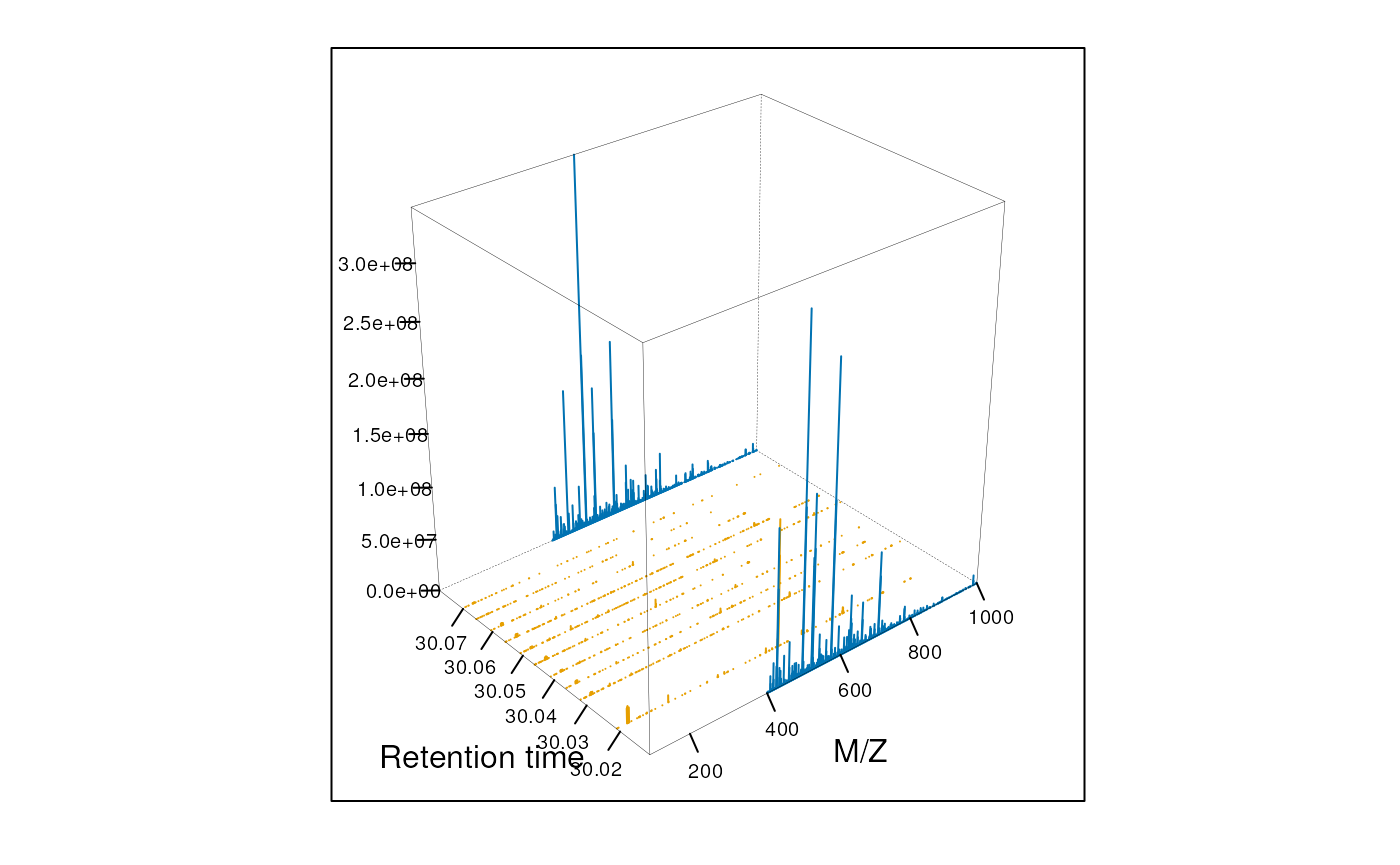#### MS barcoding

par(mar=c(4,1,1,1))
image(t(matrix(hd$msLevel, 1, nrow(hd))), xlab="Retention time", xaxt="n", yaxt="n", col=c("black","steelblue")) k <- round(range(hd$retentionTime) / 60)
nk <- 5
axis(side=1, at=seq(0,1,1/nk), labels=seq(k,k,k/nk))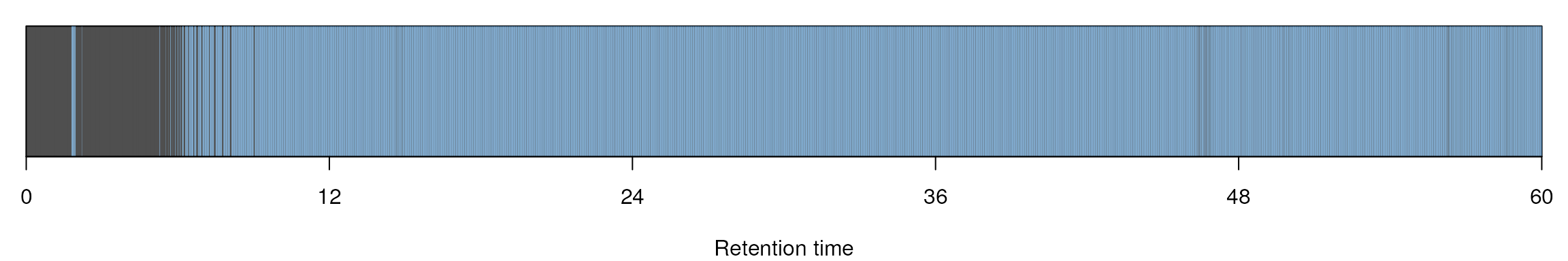#### Animation

The following animation scrolls over 5 minutes of retention time for a MZ range between 521 and 523.

library("animation")
an1 <- function() {
for (i in seq(0, 5, 0.2)) {
rtsel <- hd$retentionTime[ms1] / 60 > (30 + i) & hd$retentionTime[ms1] / 60 < (35 + i)
M <- MSmap(ms, ms1[rtsel], 521, 523, .005, hd)
M@map[msMap(M) == 0] <- NA
print(plot3D(M, rgl = FALSE))
}
}

saveGIF(an1(), movie.name = "msanim1.gif")
knitr::include_graphics("figures/msanim1.gif")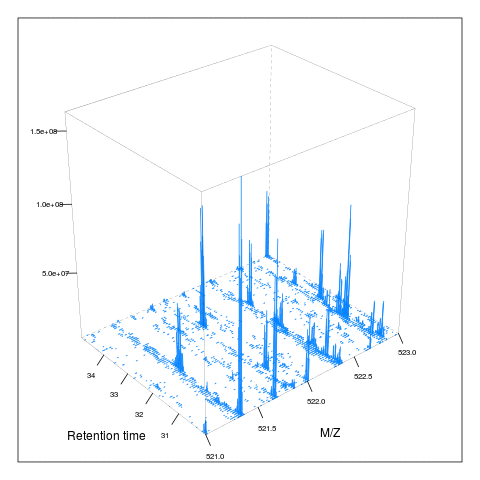The code chunk below scrolls of a slice of retention times while keeping the retention time constant between 30 and 35 minutes.

an2 <- function() {
for (i in seq(0, 2.5, 0.1)) {
rtsel <- hd$retentionTime[ms1] / 60 > 30 & hd$retentionTime[ms1] / 60 < 35
mz1 <- 520 + i
mz2 <- 522 + i
M <- MSmap(ms, ms1[rtsel], mz1, mz2, .005, hd)
M@map[msMap(M) == 0] <- NA
print(plot3D(M, rgl = FALSE))
}
}

saveGIF(an2(), movie.name = "msanim2.gif")
knitr::include_graphics("figures/msanim2.gif")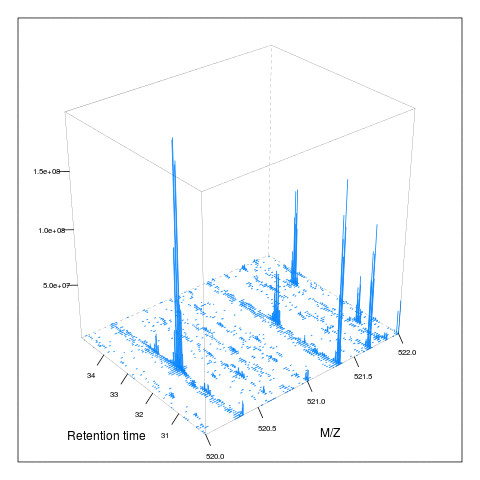### The MSnbase infrastructure

library("MSnbase")
data(itraqdata)
itraqdata2 <- pickPeaks(itraqdata, verbose = FALSE)
plot(itraqdata[], full = TRUE, reporters = iTRAQ4)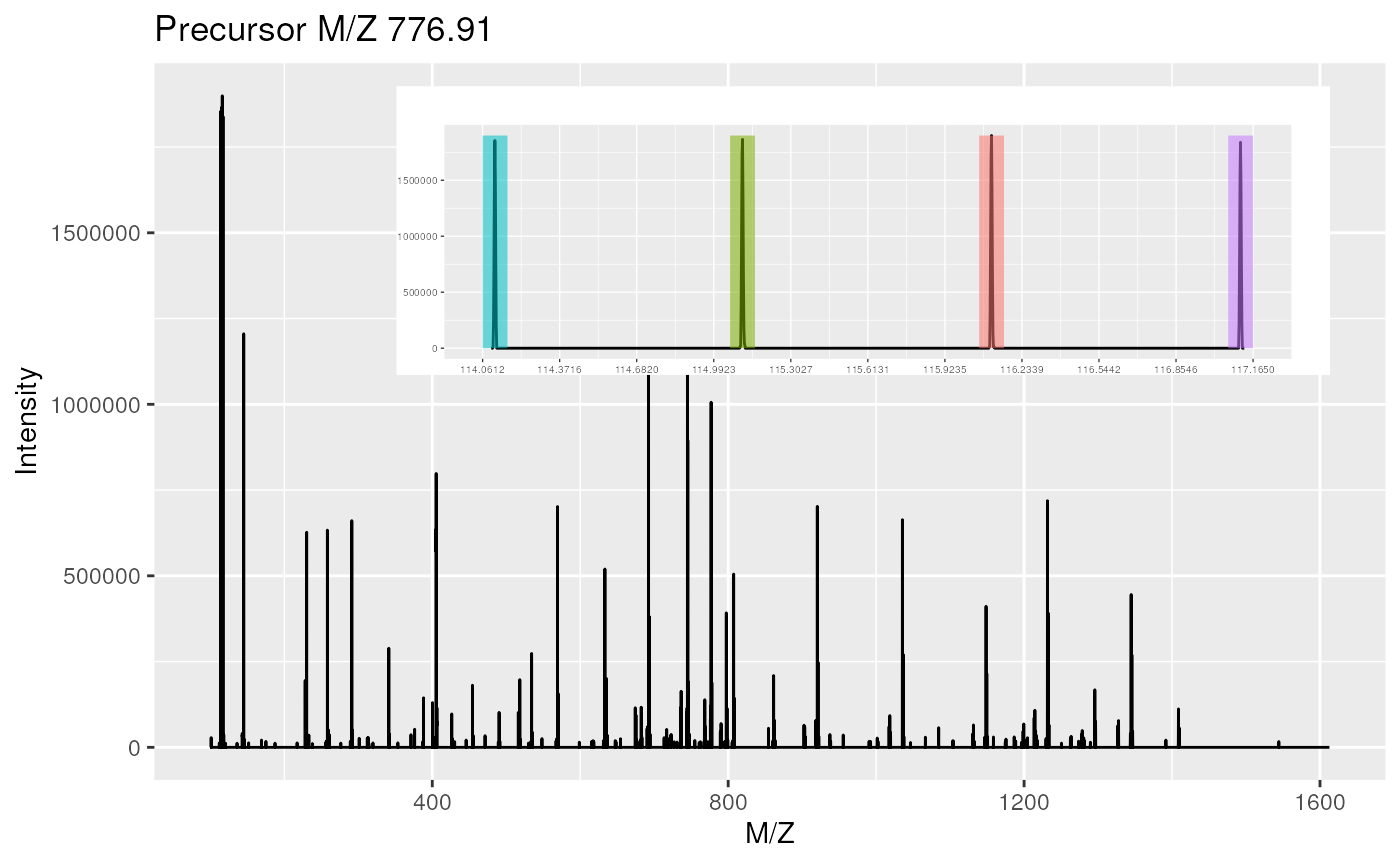par(oma = c(0, 0, 0, 0))
par(mar = c(4, 4, 1, 1))
plot(itraqdata2[], itraqdata2[], sequences = rep("IMIDLDGTENK", 2))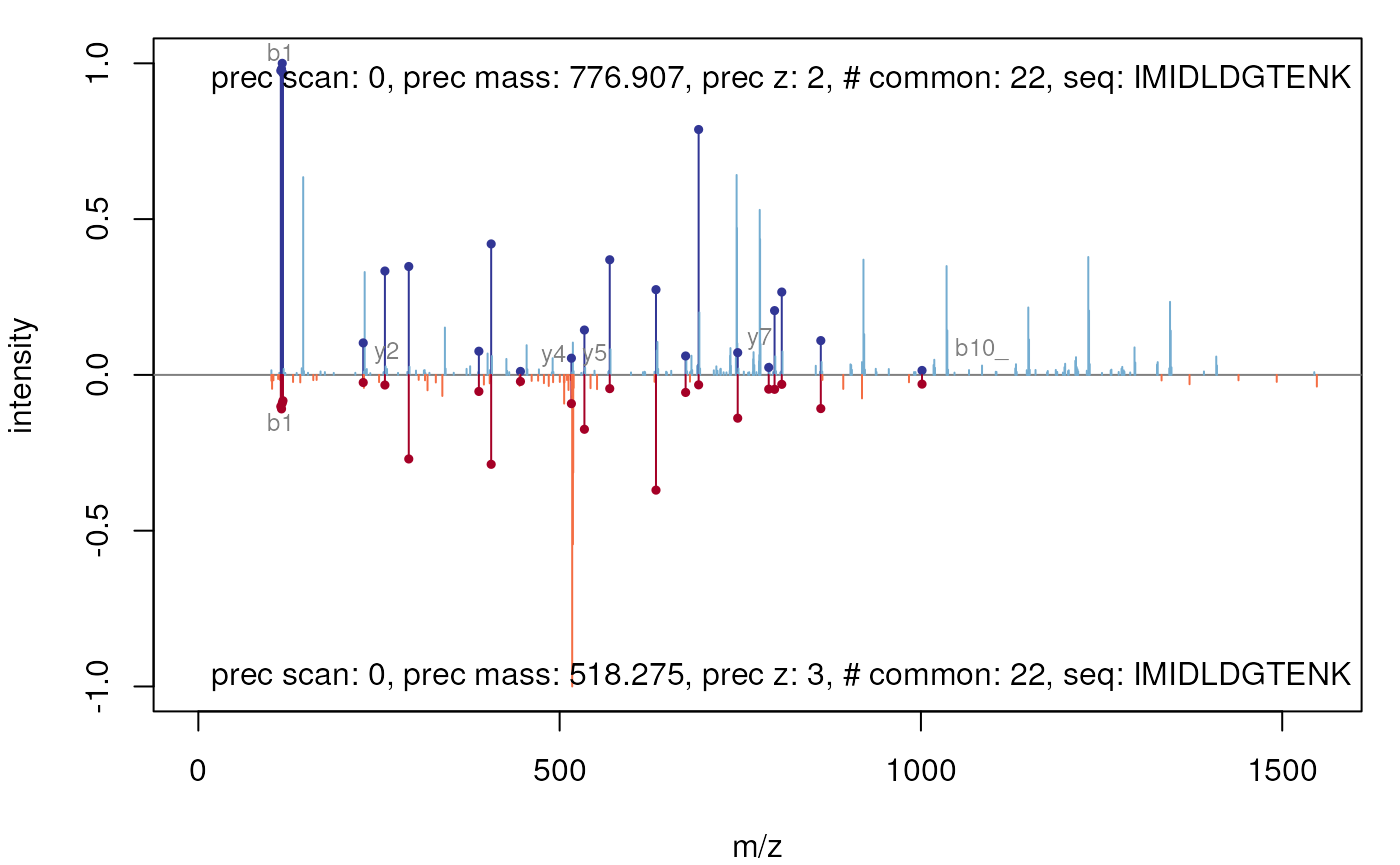### The protViz package

library("protViz")
data(msms)

fi <- fragmentIon("TAFDEAIAELDTLNEESYK")
fi.cyz <- as.data.frame(cbind(c=fi[]$c, y=fi[]$y, z=fi[]$z)) p <- peakplot("TAFDEAIAELDTLNEESYK", spec = msms[], fi = fi.cyz, itol = 0.6, ion.axes = FALSE)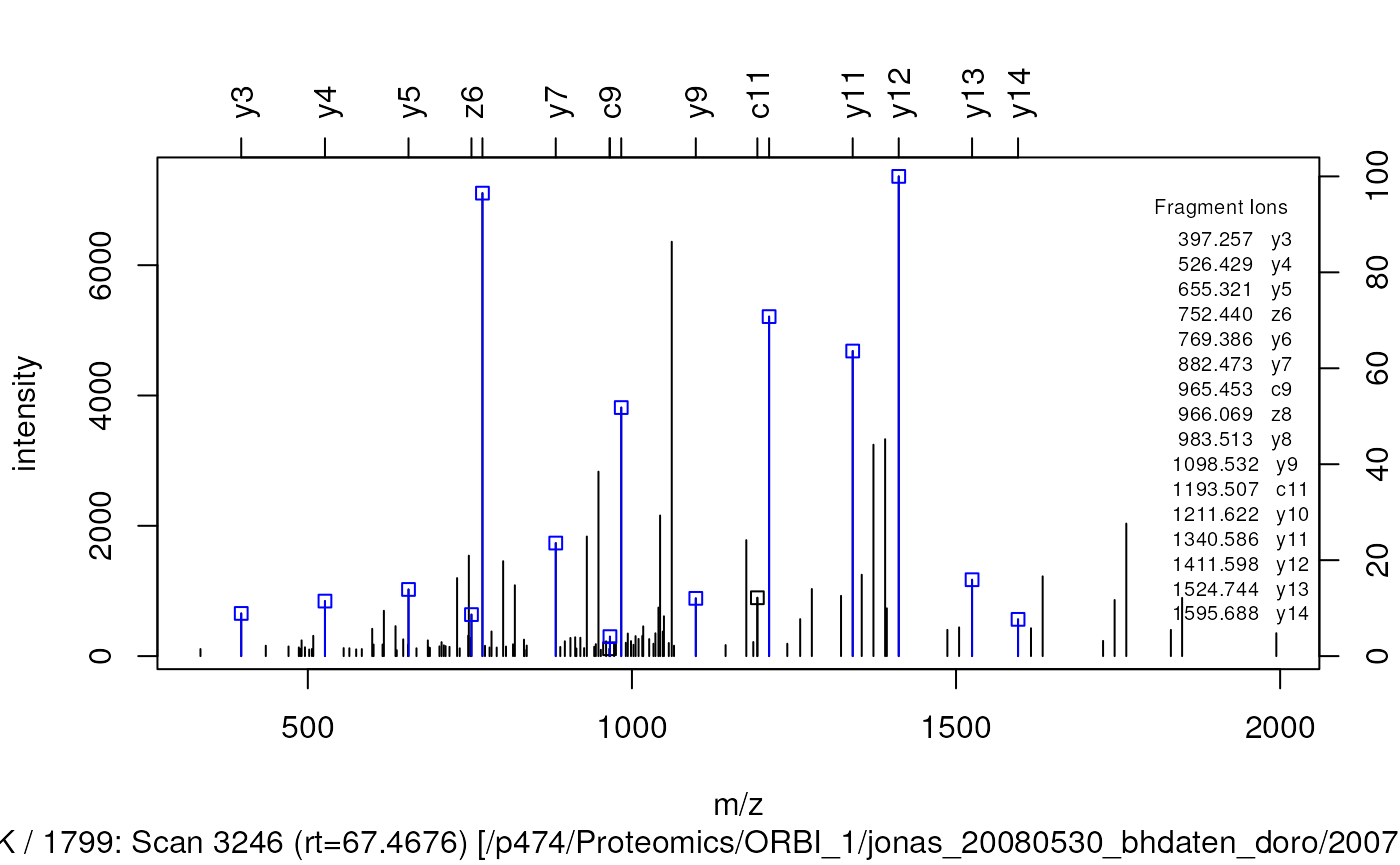The peakplot function return the annotation of the MSMS spectrum that is plotted: str(p) ## List of 7 ##$ mZ.Da.error : num [1:57] 215.3 144.27 -2.8 -17.06 2.03 ...
##  $mZ.ppm.error: num [1:57] 1808046 758830 -8306 -37724 3501 ... ##$ idx         : int [1:57] 1 1 1 3 16 24 41 52 67 88 ...
##  $label : chr [1:57] "c1" "c2" "c3" "c4" ... ##$ score       : num -1
##  $sequence : chr "TAFDEAIAELDTLNEESYK" ##$ fragmentIon :'data.frame':    19 obs. of  3 variables:
##   ..$c: num [1:19] 119 190 337 452 581 ... ## ..$ y: num [1:19] 147 310 397 526 655 ...
##   ..$z: num [1:19] 130 293 380 509 638 ... ### Preprocessing of MALDI-MS spectra The following code chunks demonstrate the usage of the mass spectrometry preprocessing and plotting routines in the r CRANpkg("MALDIquant") package. MALDIquant uses the traditional graphics system. Therefore MALDIquant overloads the traditional functions plot, lines and points for its own data types. These data types represents spectrum and peak lists as S4 classes. Please see the MALDIquant vignette and the corresponding website for more details. After loading some example data a simple plot draws the raw spectrum. library("MALDIquant") data("fiedler2009subset", package="MALDIquant") plot(fiedler2009subset[])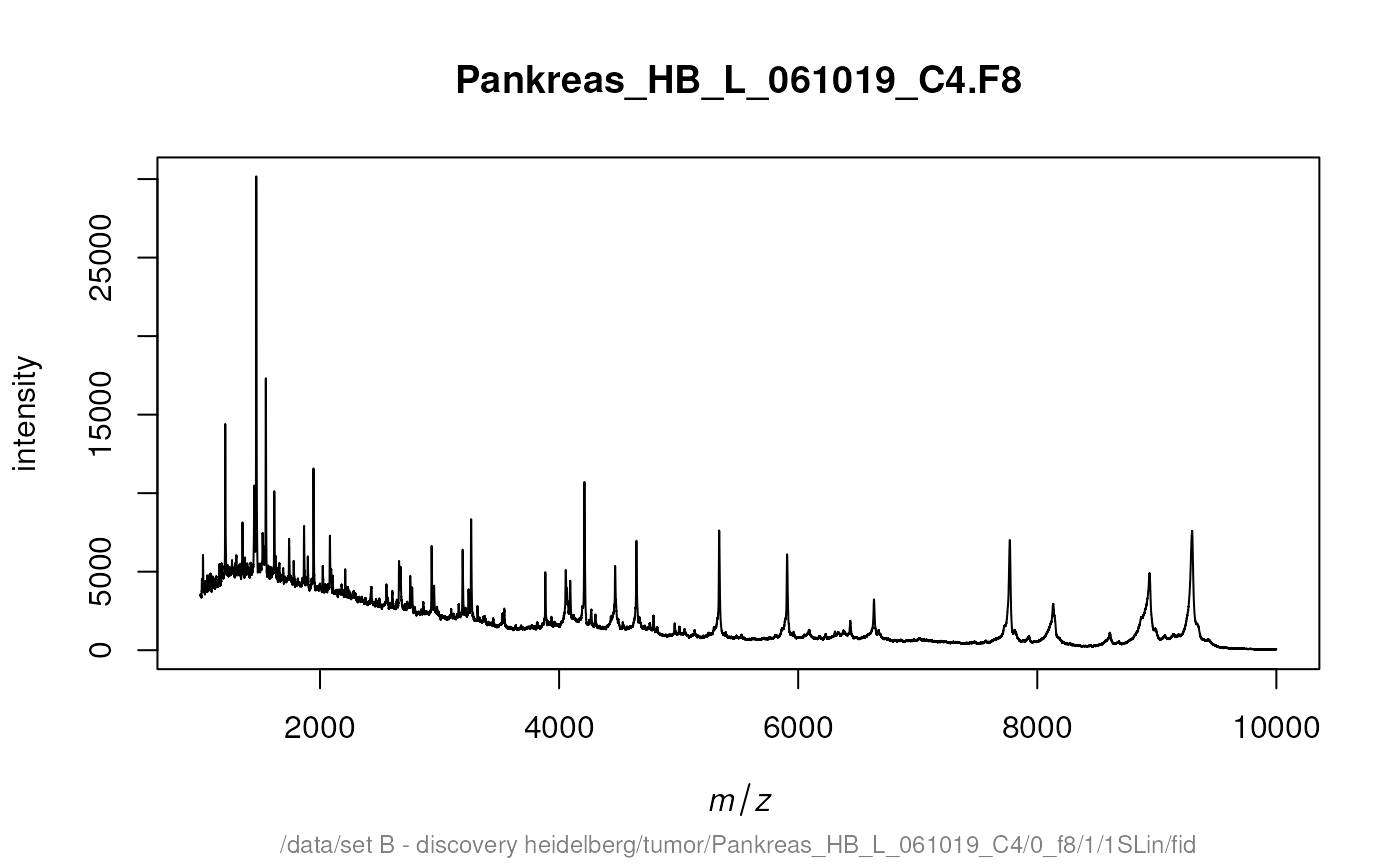After some preprocessing, namely variance stabilization and smoothing, we use lines to draw our baseline estimate in our processed spectrum. transformedSpectra <- transformIntensity(fiedler2009subset, method = "sqrt") smoothedSpectra <- smoothIntensity(transformedSpectra, method = "SavitzkyGolay") plot(smoothedSpectra[]) lines(estimateBaseline(smoothedSpectra[]), lwd = 2, col = "red")After removing the background removal we could use plot again to draw our baseline corrected spectrum. rbSpectra <- removeBaseline(smoothedSpectra) plot(rbSpectra[])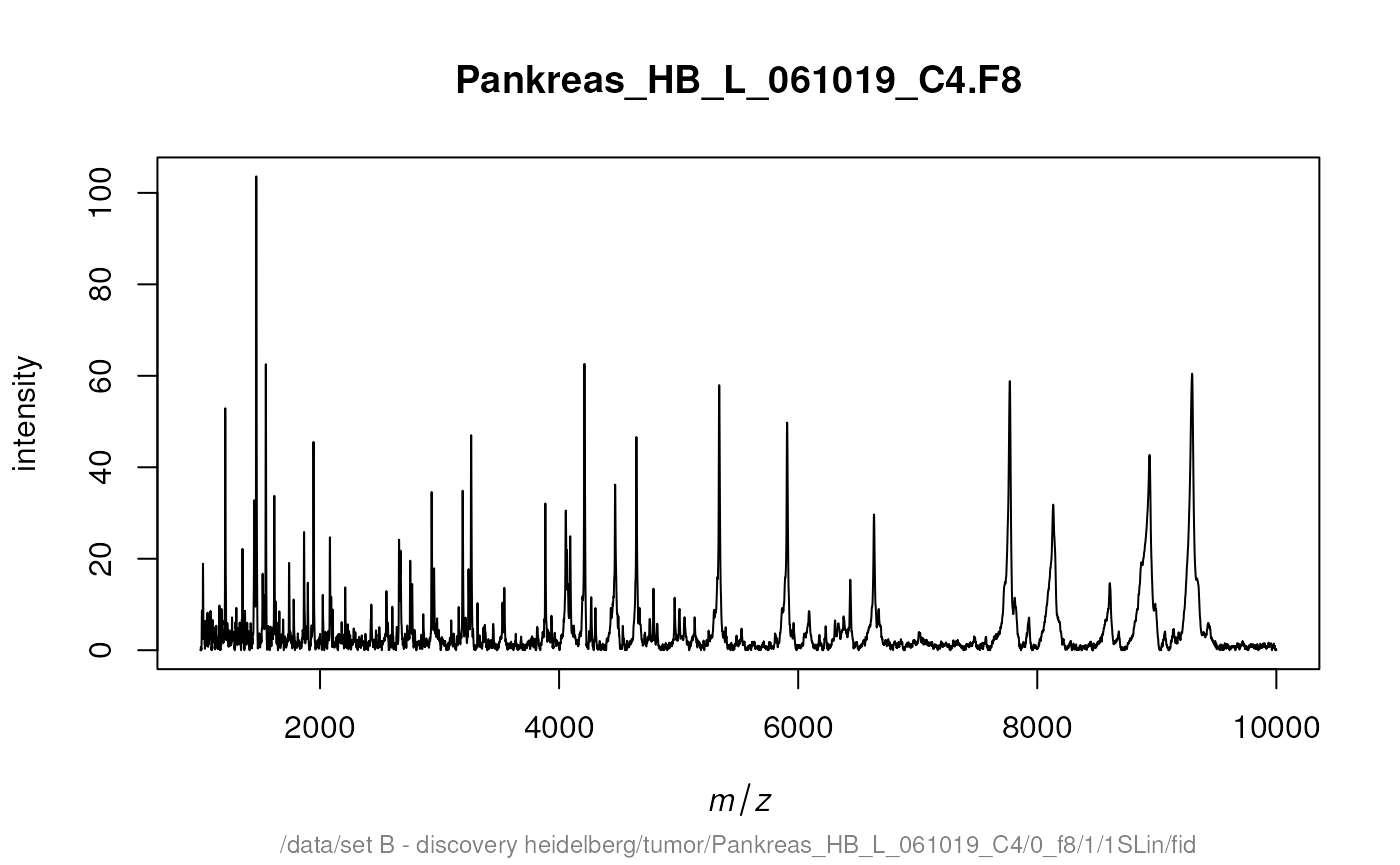detectPeaks returns a MassPeaks object that offers the same traditional graphics functions. The next code chunk demonstrates how to mark the detected peaks in a spectrum. cbSpectra <- calibrateIntensity(rbSpectra, method = "TIC") peaks <- detectPeaks(cbSpectra, SNR = 5) plot(cbSpectra[]) points(peaks[], col = "red", pch = 4, lwd = 2)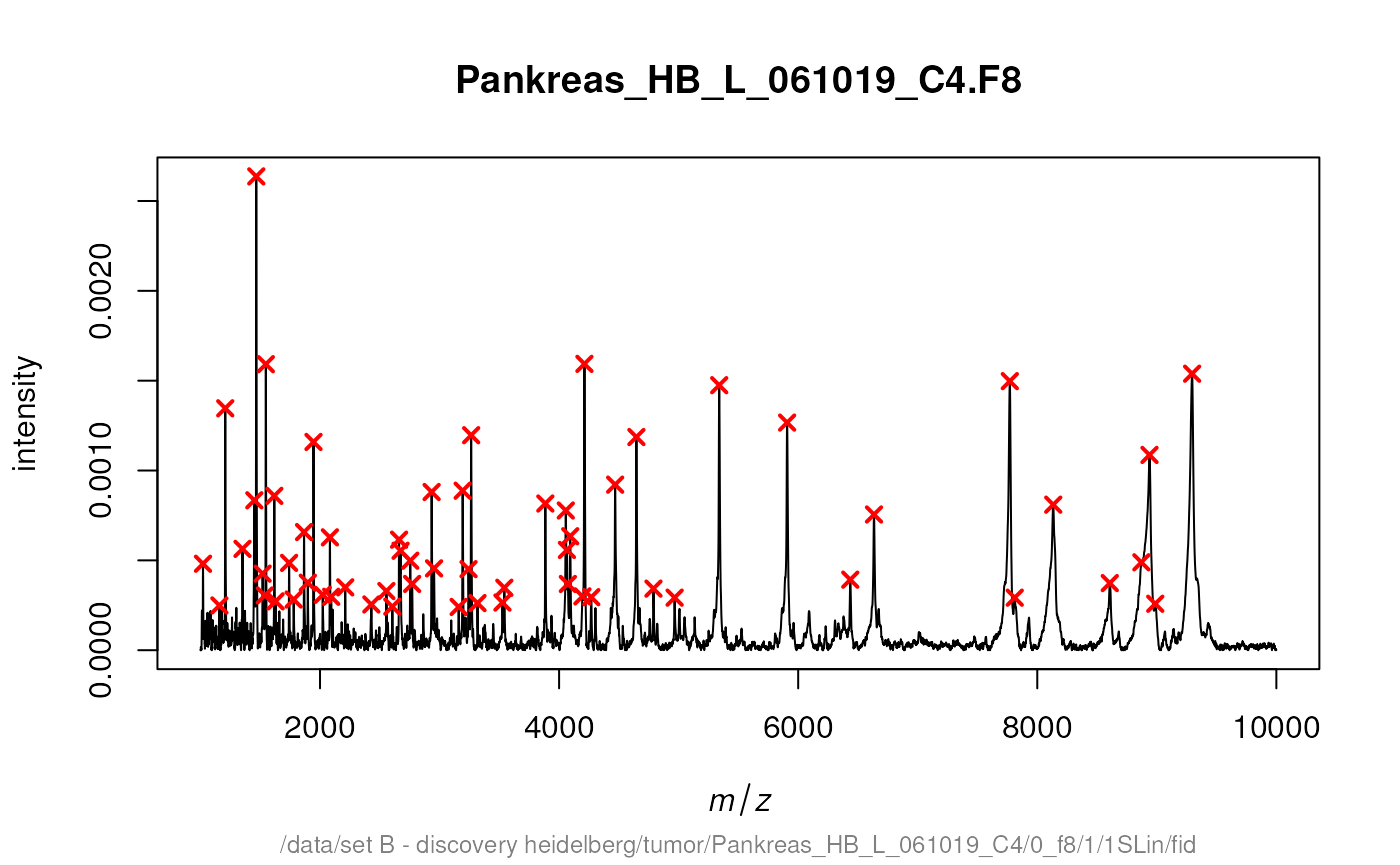Additional there is a special function labelPeaks that allows to draw the M/Z values above the corresponding peaks. Next we mark the 5 top peaks in the spectrum. top5 <- intensity(peaks[]) %in% sort(intensity(peaks[]), decreasing = TRUE)[1:5] labelPeaks(peaks[], index = top5, avoidOverlap = TRUE)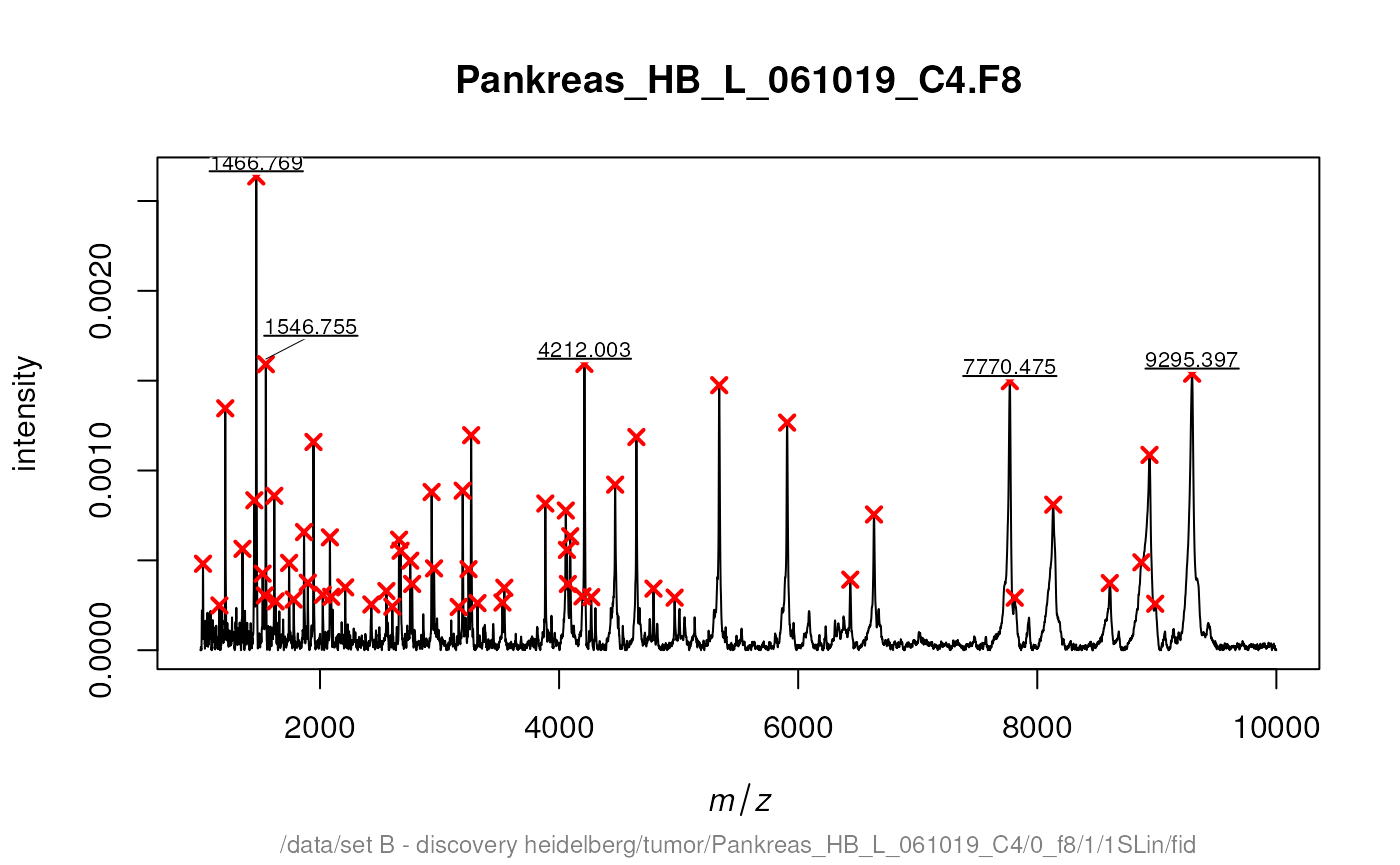Often multiple spectra have to be recalibrated to be comparable. Therefore MALDIquant warps the spectra according to so called reference or landmark peaks. For debugging the determineWarpingFunctions function offers some warping plots. Here we show only the last 4 plots: par(mfrow = c(2, 2)) warpingFunctions <- determineWarpingFunctions(peaks, tolerance = 0.001, plot = TRUE, plotInteractive = TRUE)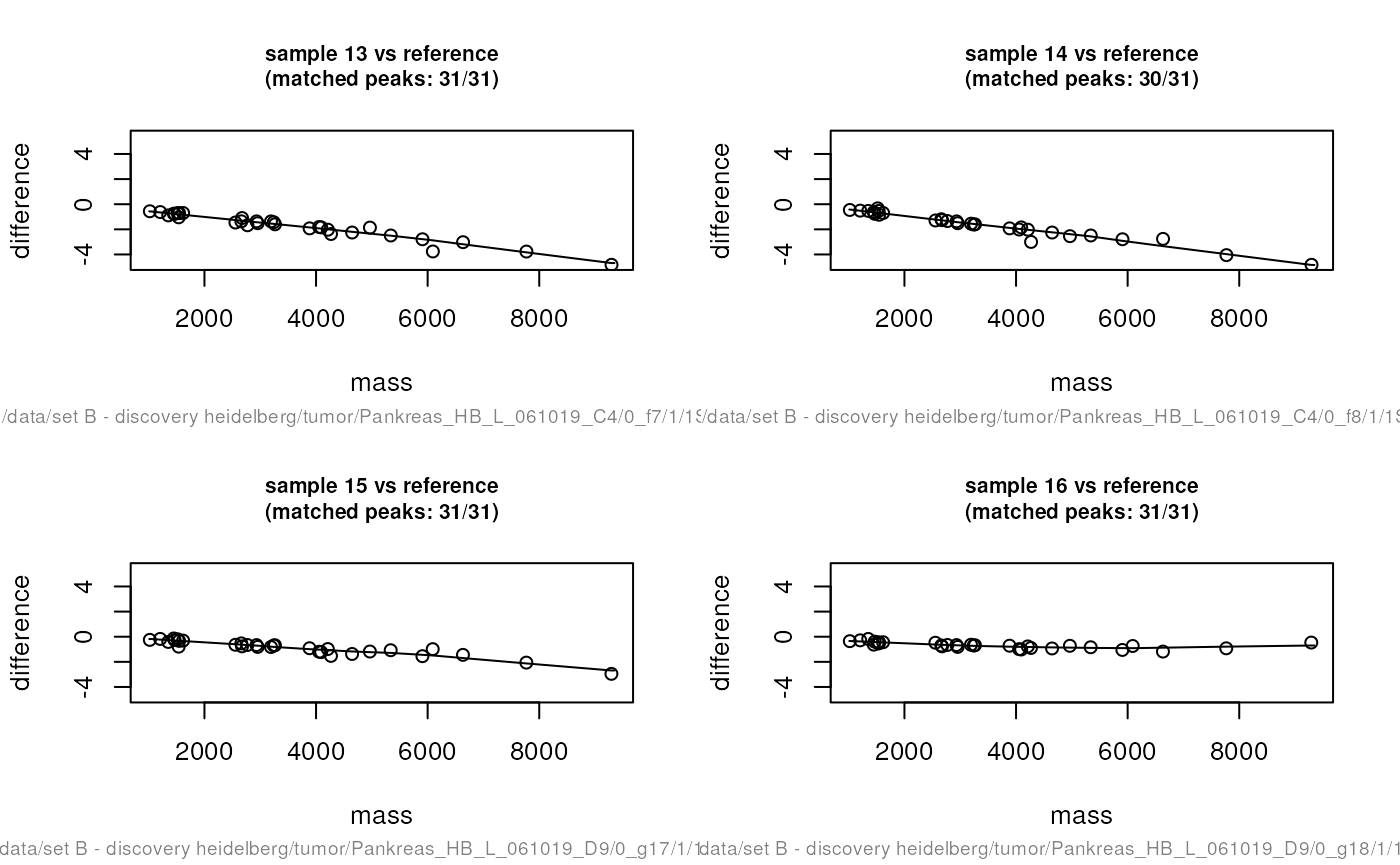par(mfrow = c(1, 1)) warpedSpectra <- warpMassSpectra(cbSpectra, warpingFunctions) warpedPeaks <- warpMassPeaks(peaks, warpingFunctions) In the next code chunk we visualise the need and the effect of the recalibration. sel <- c(2, 10, 14, 16) xlim <- c(4180, 4240) ylim <- c(0, 1.9e-3) lty <- c(1, 4, 2, 6) par(mfrow = c(1, 2)) plot(cbSpectra[], xlim = xlim, ylim = ylim, type = "n") for (i in seq(along = sel)) { lines(peaks[[sel[i]]], lty = lty[i], col = i) lines(cbSpectra[[sel[i]]], lty = lty[i], col = i) } plot(cbSpectra[], xlim = xlim, ylim = ylim, type = "n") for (i in seq(along = sel)) { lines(warpedPeaks[[sel[i]]], lty = lty[i], col = i) lines(warpedSpectra[[sel[i]]], lty = lty[i], col = i) }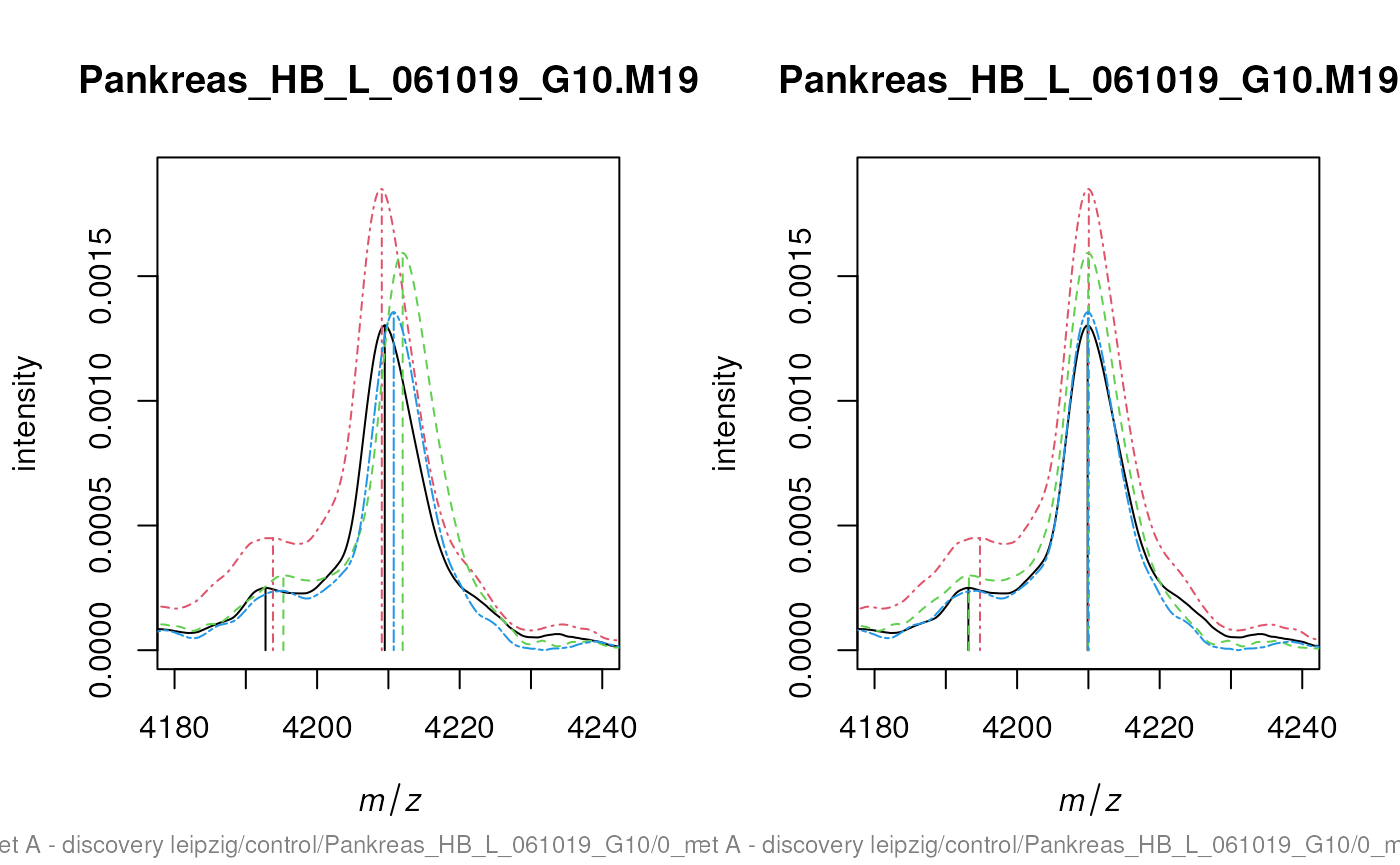par(mfrow = c(1, 1)) The code chunks above generate plots that are very similar to the figure 7 in the corresponding paper “Visualisation of proteomics data using R”. Please find the code to exactly reproduce the figure at: https://github.com/sgibb/MALDIquantExamples/blob/master/R/createFigure1_color.R ## Genomic and protein sequences These visualisations originate from the Pbase Pbase-data and mapping vignettes. ## Mass spectrometry imaging The following code chunk downloads a MALDI imaging dataset from a mouse kidney shared by Adrien Nyakas and Stefan Schurch and generates a plot with the mean spectrum and three slices of interesting M/Z regions. library("MALDIquant") library("MALDIquantForeign") spectra <- importBrukerFlex("http://files.figshare.com/1106682/MouseKidney_IMS_testdata.zip", verbose = FALSE) spectra <- smoothIntensity(spectra, "SavitzkyGolay", halfWindowSize = 8) spectra <- removeBaseline(spectra, method = "TopHat", halfWindowSize = 16) spectra <- calibrateIntensity(spectra, method = "TIC") avgSpectrum <- averageMassSpectra(spectra) avgPeaks <- detectPeaks(avgSpectrum, SNR = 5) avgPeaks <- avgPeaks[intensity(avgPeaks) > 0.0015] oldPar <- par(no.readonly = TRUE) layout(matrix(c(1,1,1,2,3,4), nrow = 2, byrow = TRUE)) plot(avgSpectrum, main = "mean spectrum", xlim = c(3000, 6000), ylim = c(0, 0.007)) lines(avgPeaks, col = "red") labelPeaks(avgPeaks, cex = 1) par(mar = c(0.5, 0.5, 1.5, 0.5)) plotMsiSlice(spectra, center = mass(avgPeaks), tolerance = 1, plotInteractive = TRUE) par(oldPar) knitr::include_graphics("figures/mqmsi-1.png")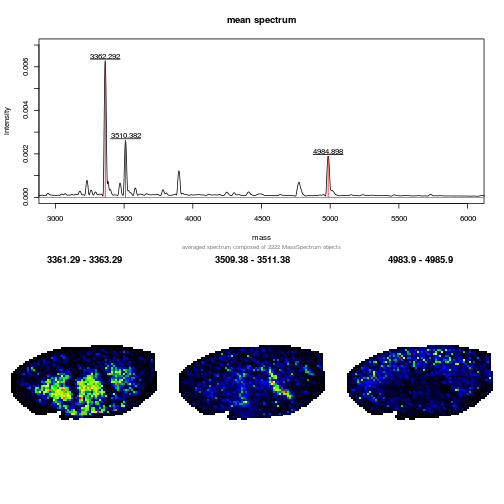### An interactive shiny app for Imaging mass spectrometry There is also an interactive MALDIquant IMS shiny app for demonstration purposes. A screen shot is displayed below. To start the application: library("shiny") runGitHub("sgibb/ims-shiny") knitr::include_graphics("figures/ims-shiny.png")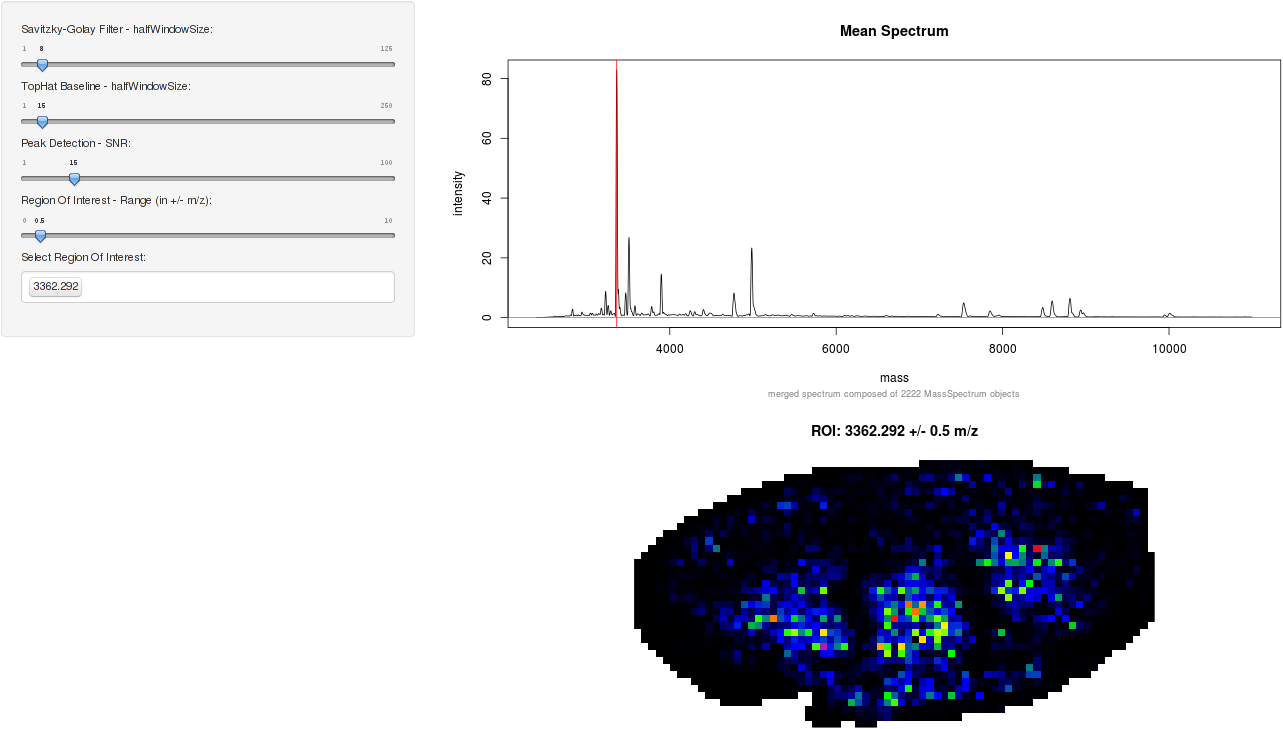## Spatial proteomics library("pRoloc") library("pRolocdata") data(tan2009r1) ## these params use class weights fn <- dir(system.file("extdata", package = "pRoloc"), full.names = TRUE, pattern = "params2.rda") load(fn) setStockcol(NULL) setStockcol(paste0(getStockcol(), 90)) w <- table(fData(tan2009r1)[, "pd.markers"]) (w <- 1/w[names(w) != "unknown"]) ## ## Cytoskeleton ER Golgi Lysosome mitochondrion ## 0.14285714 0.05000000 0.16666667 0.12500000 0.07142857 ## Nucleus Peroxisome PM Proteasome Ribosome 40S ## 0.05000000 0.25000000 0.06666667 0.09090909 0.07142857 ## Ribosome 60S ## 0.04000000 tan2009r1 <- svmClassification(tan2009r1, params2, class.weights = w, fcol = "pd.markers") ##  "pd.markers" ## Registered S3 method overwritten by 'gdata': ## method from ## reorder.factor gplots ptsze <- exp(fData(tan2009r1)$svm.scores) - 1
lout <- matrix(c(1:4, rep(5, 4)), ncol = 4, nrow = 2)
layout(lout)
cls <- getStockcol()
par(mar = c(4, 4, 1, 1))
plotDist(tan2009r1[which(fData(tan2009r1)$PLSDA == "mitochondrion"), ], markers = featureNames(tan2009r1)[which(fData(tan2009r1)$markers.orig == "mitochondrion")],
mcol = cls)
legend("topright", legend = "mitochondrion", bty = "n")
plotDist(tan2009r1[which(fData(tan2009r1)$PLSDA == "ER/Golgi"), ], markers = featureNames(tan2009r1)[which(fData(tan2009r1)$markers.orig == "ER")],
mcol = cls)
legend("topright", legend = "ER", bty = "n")
plotDist(tan2009r1[which(fData(tan2009r1)$PLSDA == "ER/Golgi"), ], markers = featureNames(tan2009r1)[which(fData(tan2009r1)$markers.orig == "Golgi")],
mcol = cls)
legend("topright", legend = "Golgi", bty = "n")
plotDist(tan2009r1[which(fData(tan2009r1)$PLSDA == "PM"), ], markers = featureNames(tan2009r1)[which(fData(tan2009r1)$markers.orig == "PM")],
mcol = cls)
legend("topright", legend = "PM", bty = "n")
plot2D(tan2009r1, fcol = "svm", cex = ptsze, method = "kpca")
addLegend(tan2009r1, where = "bottomleft", fcol = "svm", bty = "n")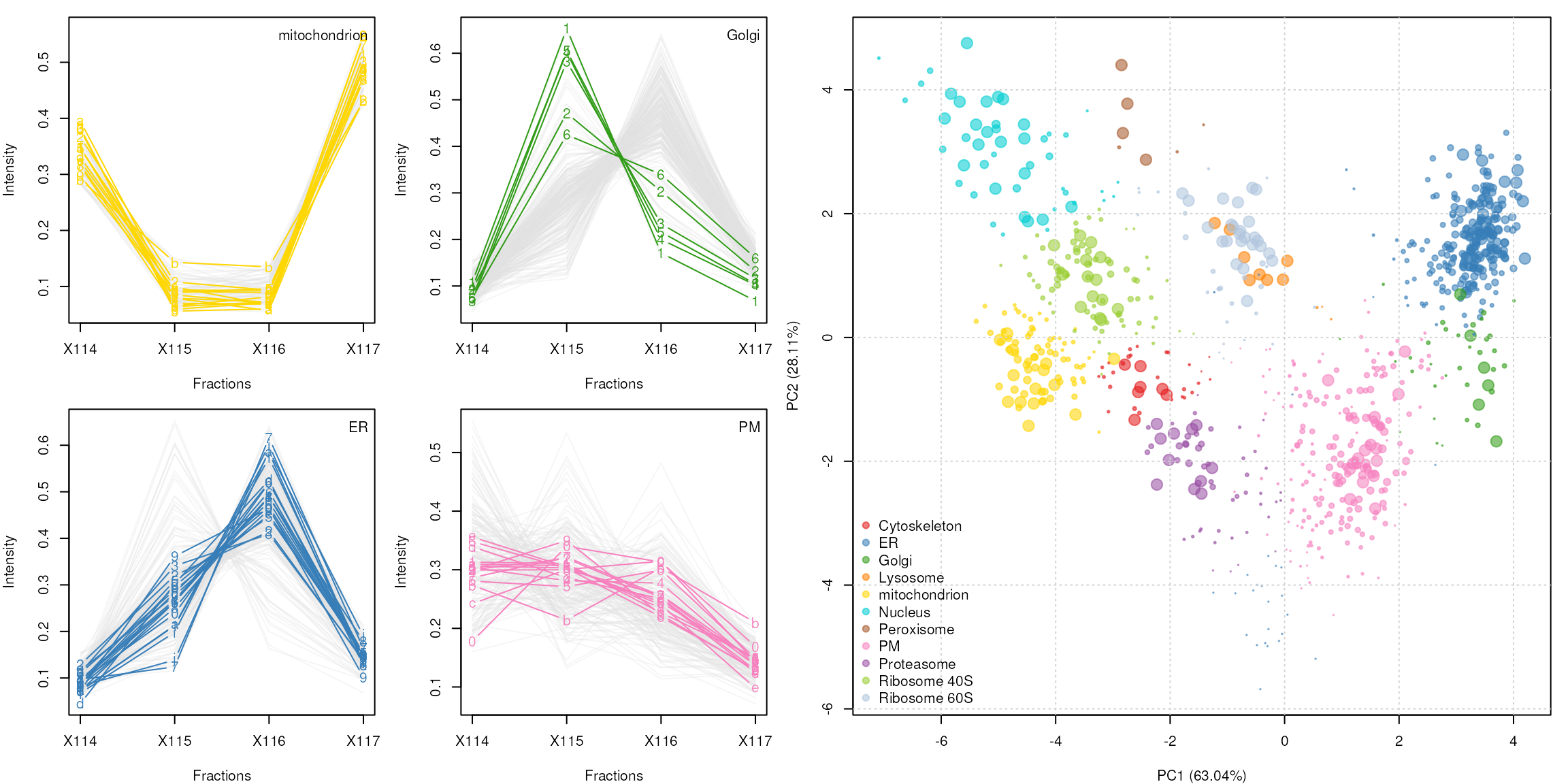See the pRoloc-tutorial vignette (pdf) from the pRoloc package for details about spatial proteomics data analysis and visualisation.

## Session information

print(sessionInfo(), locale = FALSE)
## R version 4.3.0 beta (2023-04-12 r84240)
## Platform: x86_64-pc-linux-gnu (64-bit)
## Running under: Ubuntu 22.04.2 LTS
##
## Matrix products: default
## LAPACK: /usr/lib/x86_64-linux-gnu/openblas-pthread/libopenblasp-r0.3.20.so;  LAPACK version 3.10.0
##
## attached base packages:
##  stats4    stats     graphics  grDevices utils     datasets  methods
##  base
##
## other attached packages:
##   beanplot_1.3.1         ggplot2_3.4.2          lattice_0.21-8
##   e1071_1.7-13           msmsTests_1.37.0       msmsEDA_1.37.0
##   pRolocdata_1.37.1      pRoloc_1.39.1          BiocParallel_1.33.12
##  MLInterfaces_1.79.1    cluster_2.1.4          annotate_1.77.0
##  XML_3.99-0.14          AnnotationDbi_1.61.2   IRanges_2.33.1
##  MALDIquantForeign_0.13 MALDIquant_1.22.1      RColorBrewer_1.1-3
##  xtable_1.8-4           rpx_2.7.5              knitr_1.42
##  DT_0.27                protViz_0.7.7          BiocManager_1.30.20
##  RforProteomics_1.37.4  MSnbase_2.25.2         ProtGenerics_1.31.0
##  S4Vectors_0.37.7       mzR_2.33.1             Rcpp_1.0.10
##  Biobase_2.59.0         BiocGenerics_0.45.3    BiocStyle_2.27.2
##
## loaded via a namespace (and not attached):
##    segmented_1.6-4             fs_1.6.1
##    matrixStats_0.63.0          bitops_1.0-7
##    lubridate_1.9.2             httr_1.4.5
##    doParallel_1.0.17           tools_4.3.0
##    utf8_1.2.3                  R6_2.5.1
##   lazyeval_0.2.2              withr_2.5.0
##   prettyunits_1.1.1           gridExtra_2.3
##   preprocessCore_1.61.0       cli_3.6.1
##   labeling_0.4.2              sass_0.4.5
##   mvtnorm_1.1-3               randomForest_4.7-1.1
##   proxy_0.4-27                pkgdown_2.0.7.9000
##   systemfonts_1.0.4           R.utils_2.12.2
##   parallelly_1.35.0           limma_3.55.7
##   impute_1.73.0               RSQLite_2.3.1
##   FNN_1.1.3.2                 generics_0.1.3
##   gtools_3.9.4                crosstalk_1.2.0
##   dplyr_1.1.2                 dendextend_1.17.1
##   Matrix_1.5-4                fansi_1.0.4
##   R.methodsS3_1.8.2           lifecycle_1.0.3
##   yaml_2.3.7                  edgeR_3.41.6
##   SummarizedExperiment_1.29.1 gplots_3.1.3
##   biocViews_1.67.3            recipes_1.0.5
##   qvalue_2.31.1               BiocFileCache_2.7.2
##   grid_4.3.0                  blob_1.2.4
##   gdata_2.18.0.1              crayon_1.5.2
##   KEGGREST_1.39.0             pillar_1.9.0
##   GenomicRanges_1.51.4        future.apply_1.10.0
##   lpSolve_5.6.18              codetools_0.2-19
##   glue_1.6.2                  pcaMethods_1.91.0
##   data.table_1.14.8           vctrs_0.6.2
##   png_0.1-8                   gtable_0.3.3
##   kernlab_0.9-32              cachem_1.0.7
##   gower_1.0.1                 xfun_0.39
##   prodlim_2023.03.31          coda_0.19-4
##   survival_3.5-5              ncdf4_1.21
##   timeDate_4022.108           iterators_1.0.14
##   hardhat_1.3.0               lava_1.7.2.1
##   ellipsis_0.3.2              ipred_0.9-14
##   nlme_3.1-162                bit64_4.0.5
##   progress_1.2.2              filelock_1.0.2
##   LaplacesDemon_16.1.6        GenomeInfoDb_1.35.17
##   rprojroot_2.0.3             bslib_0.4.2
##   affyio_1.69.0               KernSmooth_2.23-20
##   rpart_4.1.19                colorspace_2.1-0
##   DBI_1.1.3                   nnet_7.3-18
##   tidyselect_1.2.0            bit_4.0.5
##   compiler_4.3.0              curl_5.0.0
##   graph_1.77.3                xml2_1.3.3
##   desc_1.4.2                  DelayedArray_0.25.0
##  plotly_4.10.1               bookdown_0.33
##  scales_1.2.1                caTools_1.18.2
##  hexbin_1.28.3               affy_1.77.0
##  RBGL_1.75.1                 rappdirs_0.3.3
##  stringr_1.5.0               digest_0.6.31
##  mixtools_2.0.0              rmarkdown_2.21
##  XVector_0.39.0              htmltools_0.5.5
##  pkgconfig_2.0.3             base64enc_0.1-3
##  MatrixGenerics_1.11.1       highr_0.10
##  dbplyr_2.3.2                fastmap_1.1.1
##  rlang_1.1.0                 htmlwidgets_1.6.2
##  farver_2.1.1                jquerylib_0.1.4
##  jsonlite_1.8.4              mclust_6.0.0
##  mzID_1.37.0                 ModelMetrics_1.2.2.2
##  R.oo_1.25.0                 RCurl_1.98-1.12
##  magrittr_2.0.3              GenomeInfoDbData_1.2.10
##  munsell_0.5.0               viridis_0.6.2
##  MsCoreUtils_1.11.6          vsn_3.67.0
##  stringi_1.7.12              pROC_1.18.0
##  zlibbioc_1.45.0             MASS_7.3-58.4
##  caret_6.0-94                sampling_2.9